# Selina Solutions for Class 9 Physics Chapter 7 - Reflection of Light

Selina Solutions for Class 9 Physics Chapter 7 Reflection of Light guides students towards a complete guide for all textbook questions, solved to the point, providing in-depth information in the best possible schema. ICSE Class 9 students are supplied with the best possible study tool in the form of Selina solutions, providing step-by-step solutions.

For a better understanding and students’ ease, questions are provided along with answers and diagrams so that students do not have to go back to the textbook. Selina solutions are completely reliable as answers are framed with the information provided in the textbook only. This way students can rest assured from the examination point of view.

One of the other advantages of referring to ICSE Class 9 Selina Physics solutions is that students can analyze the answering methodology and pick up the tactics to answer in the examination where time is an important factor.

### Access Answers of Physics Selina Solutions class 9 Chapter 7 – Reflection of Light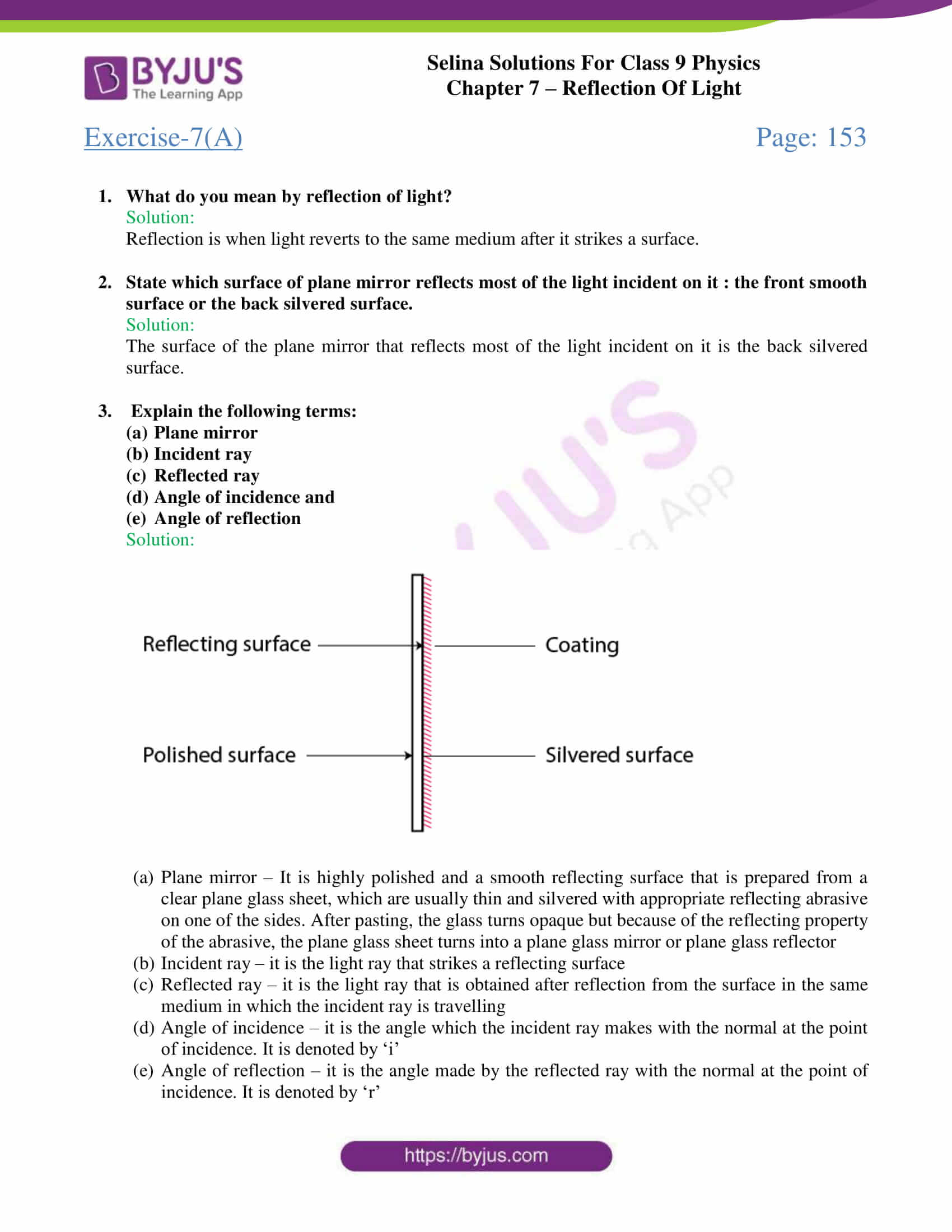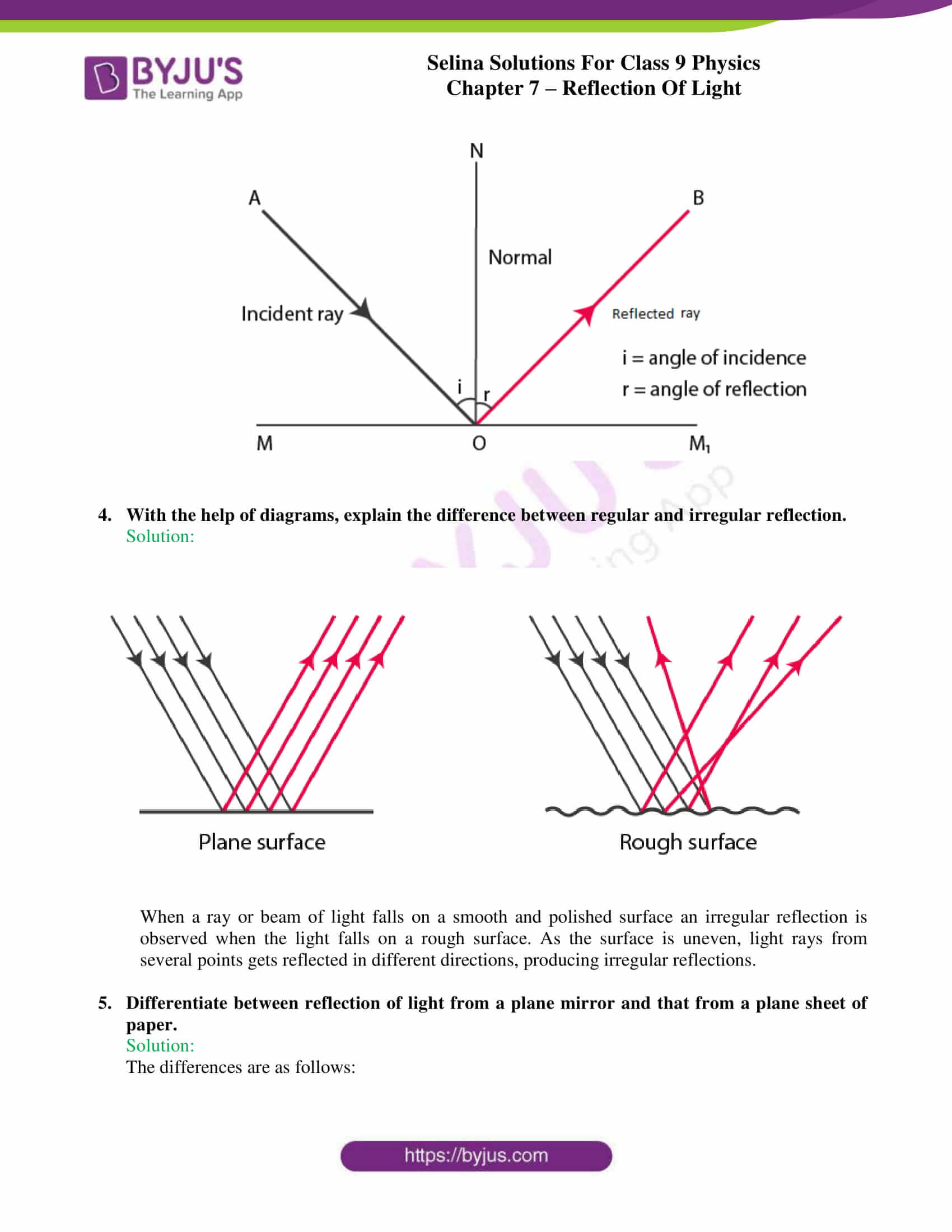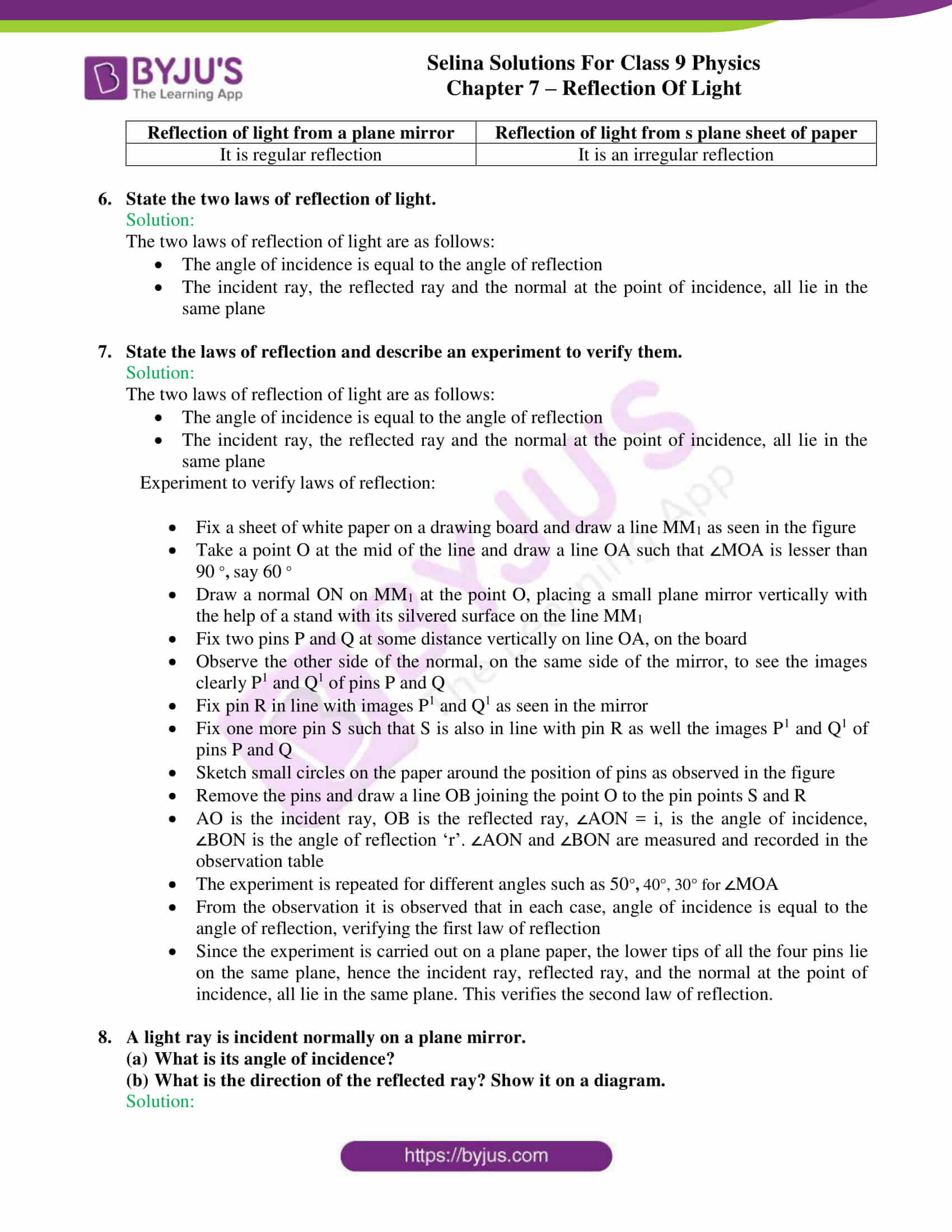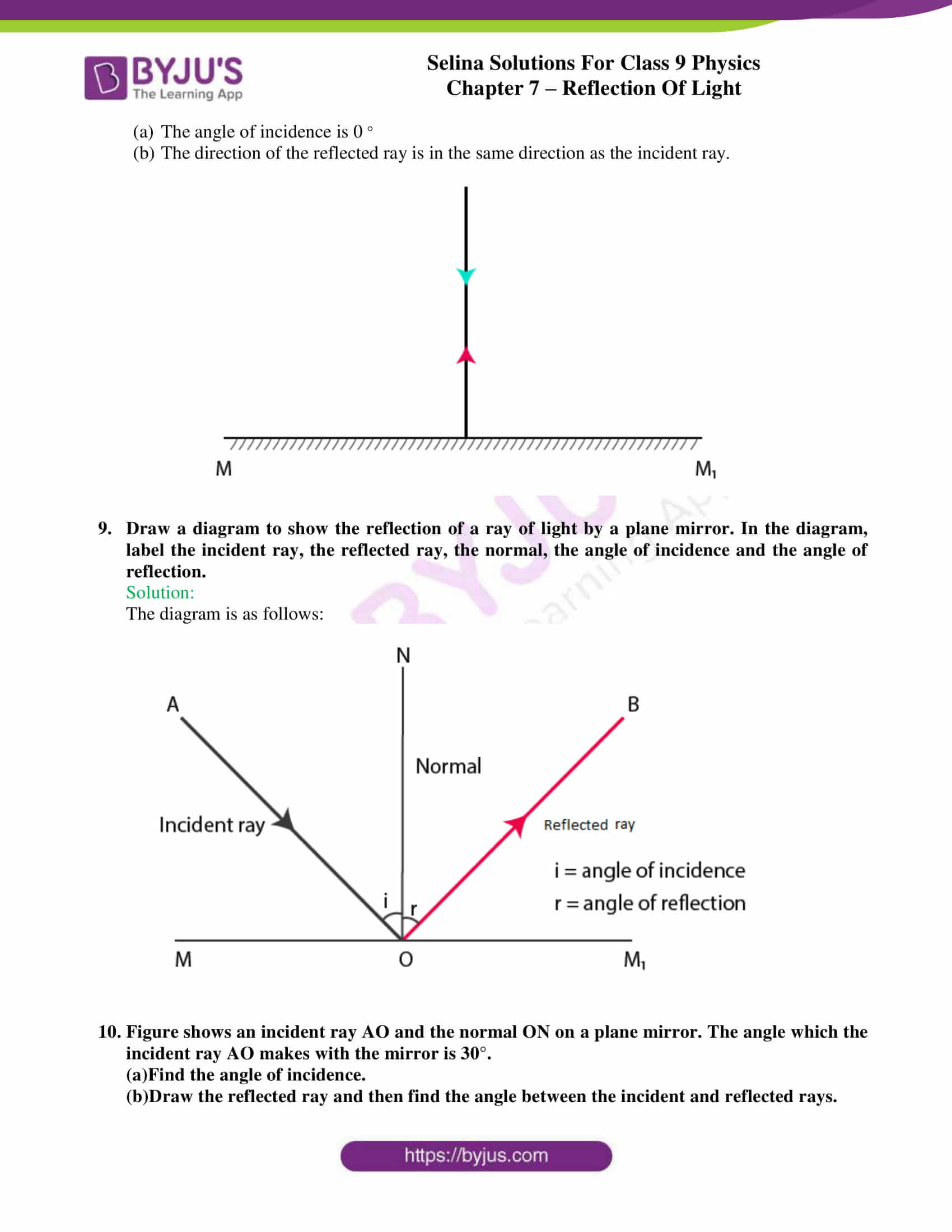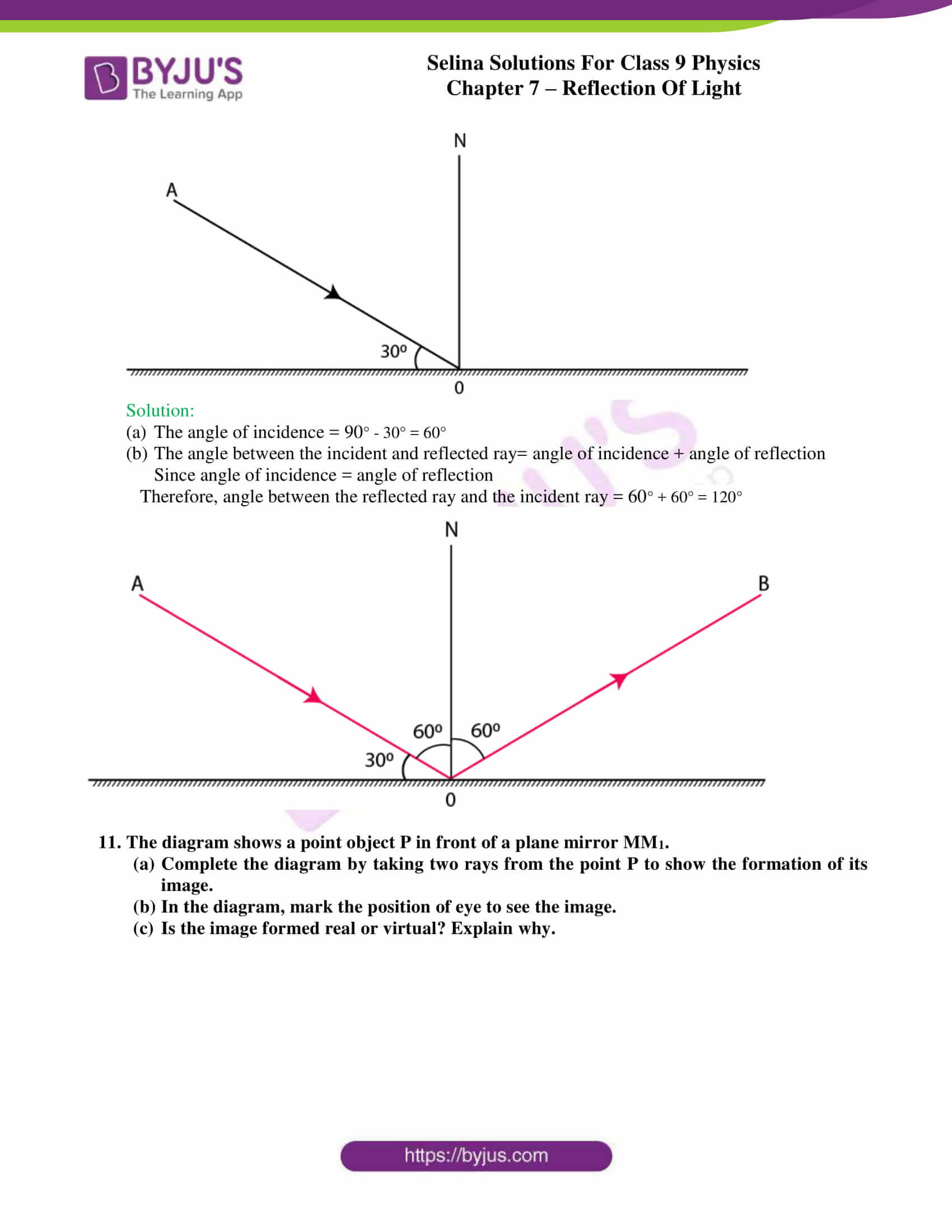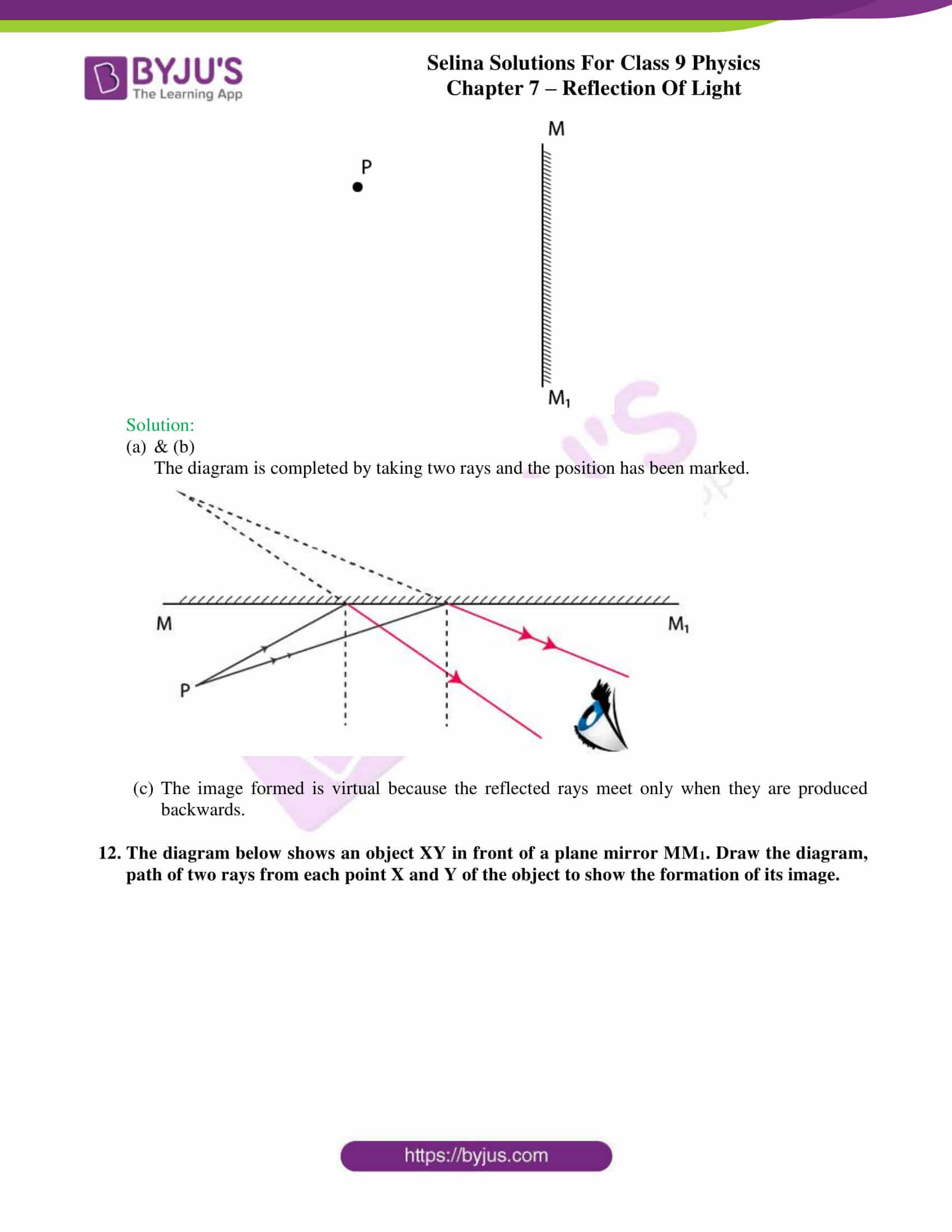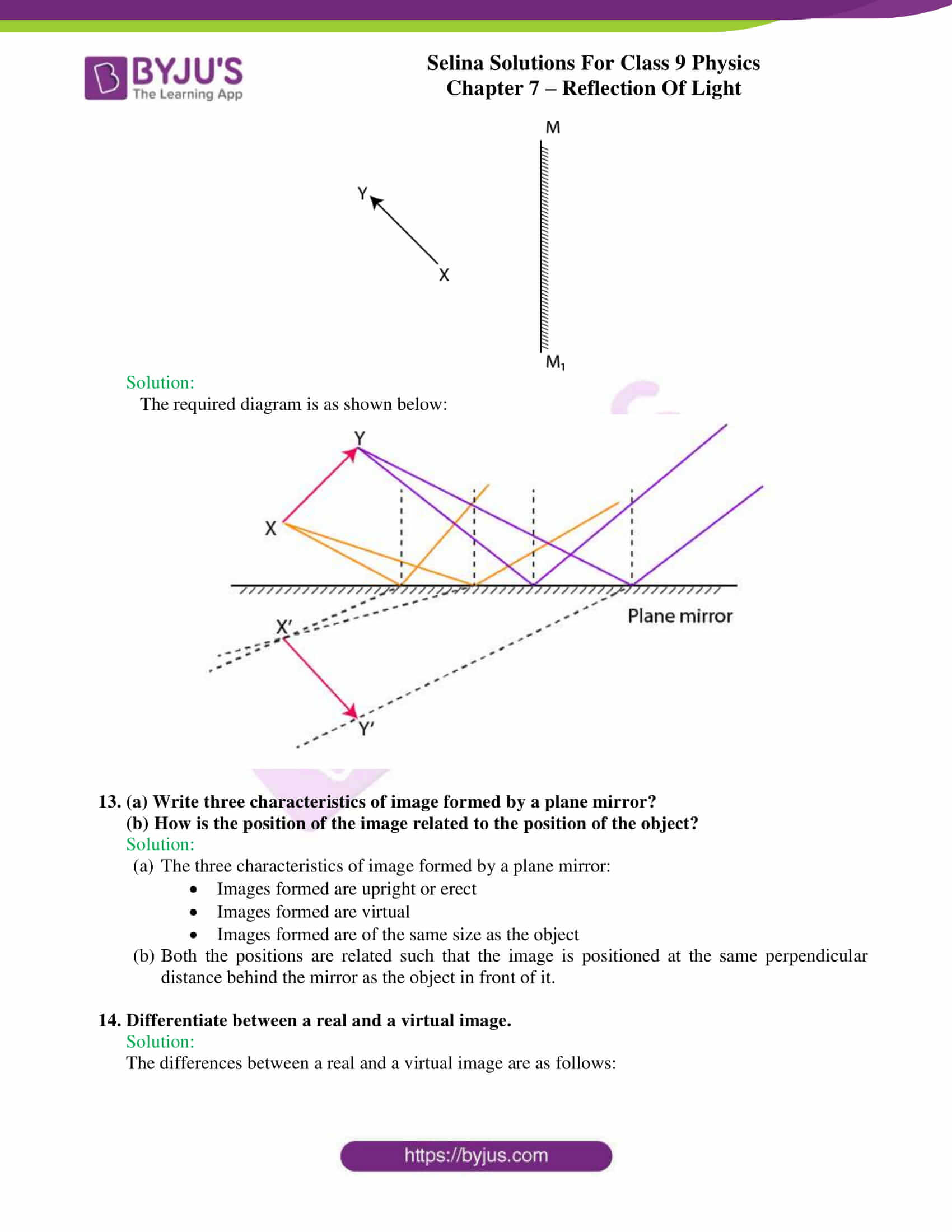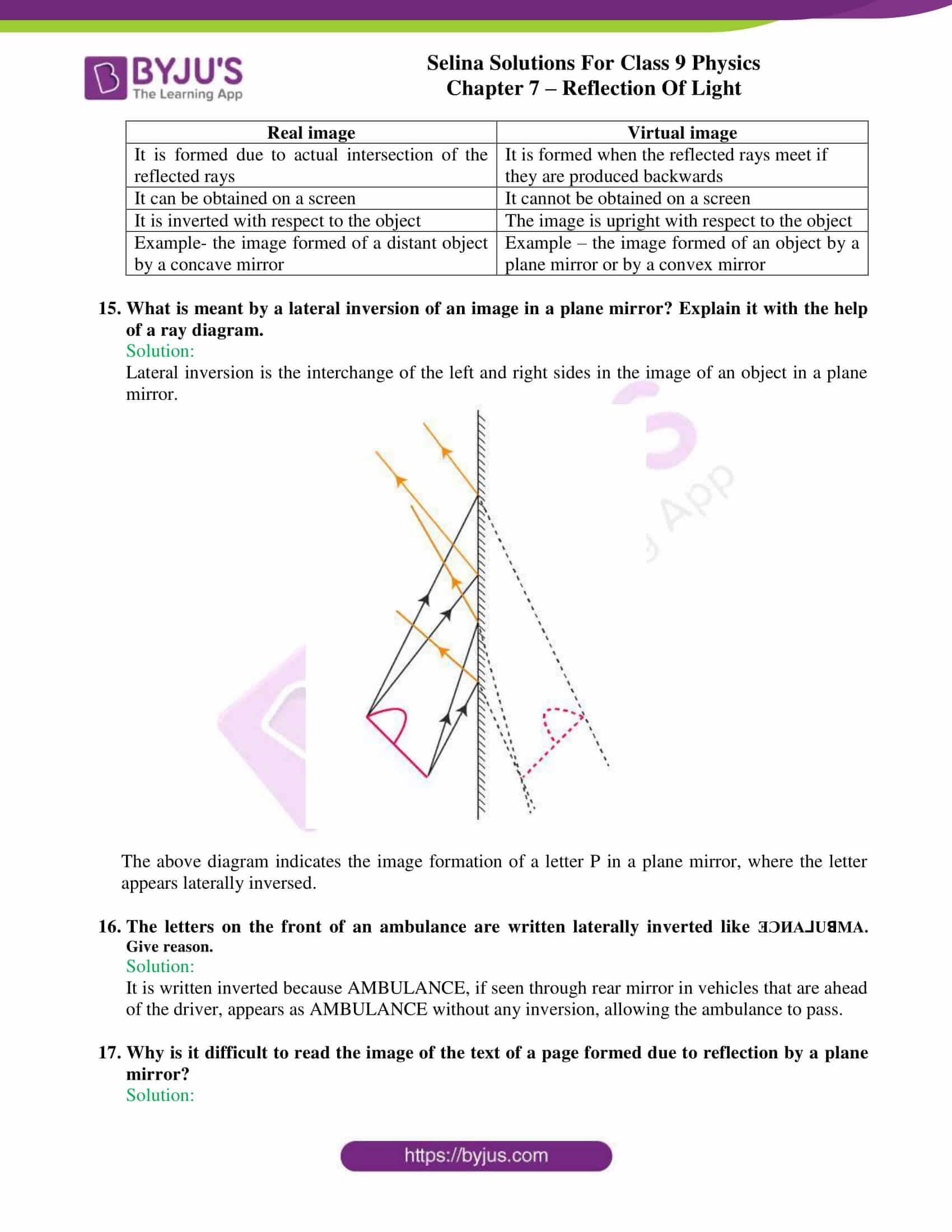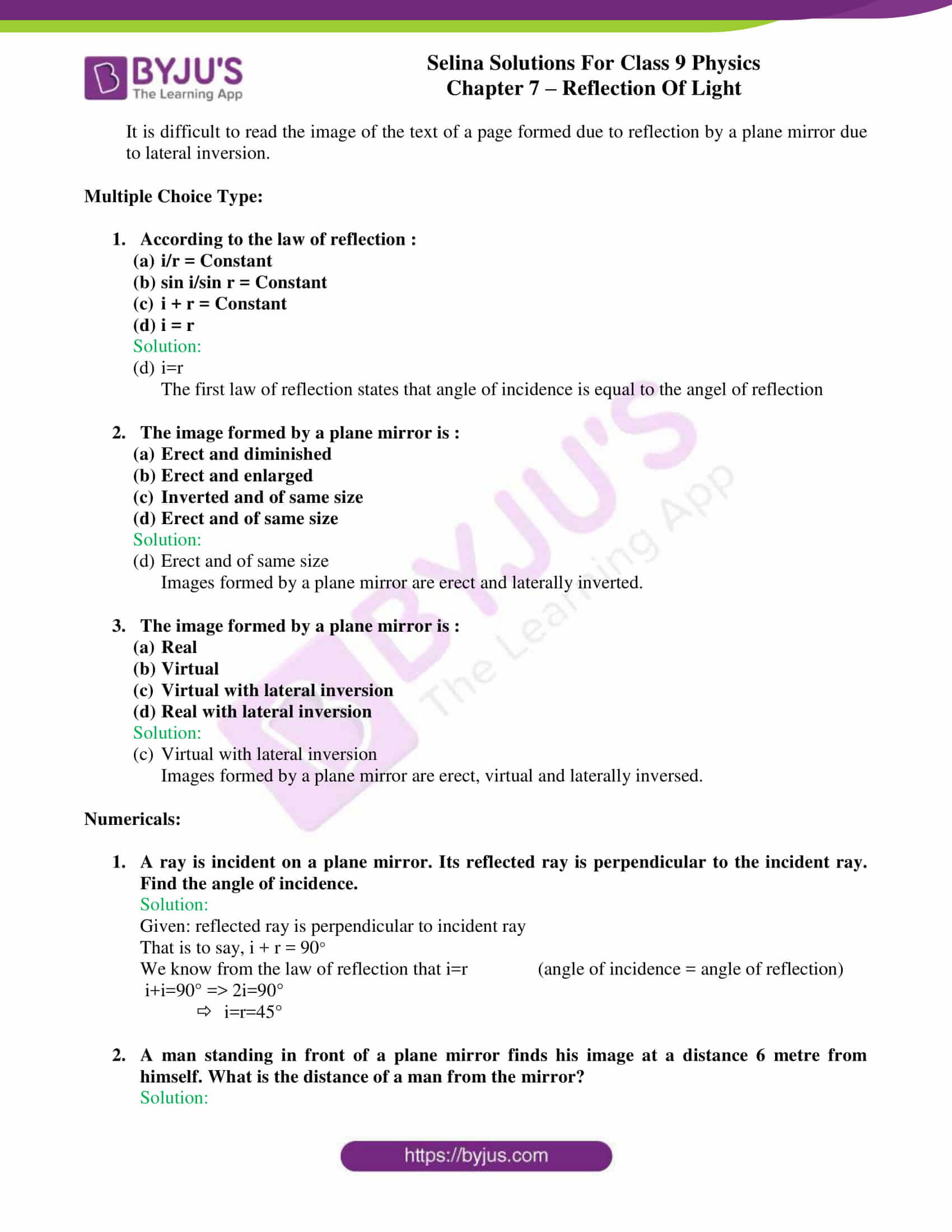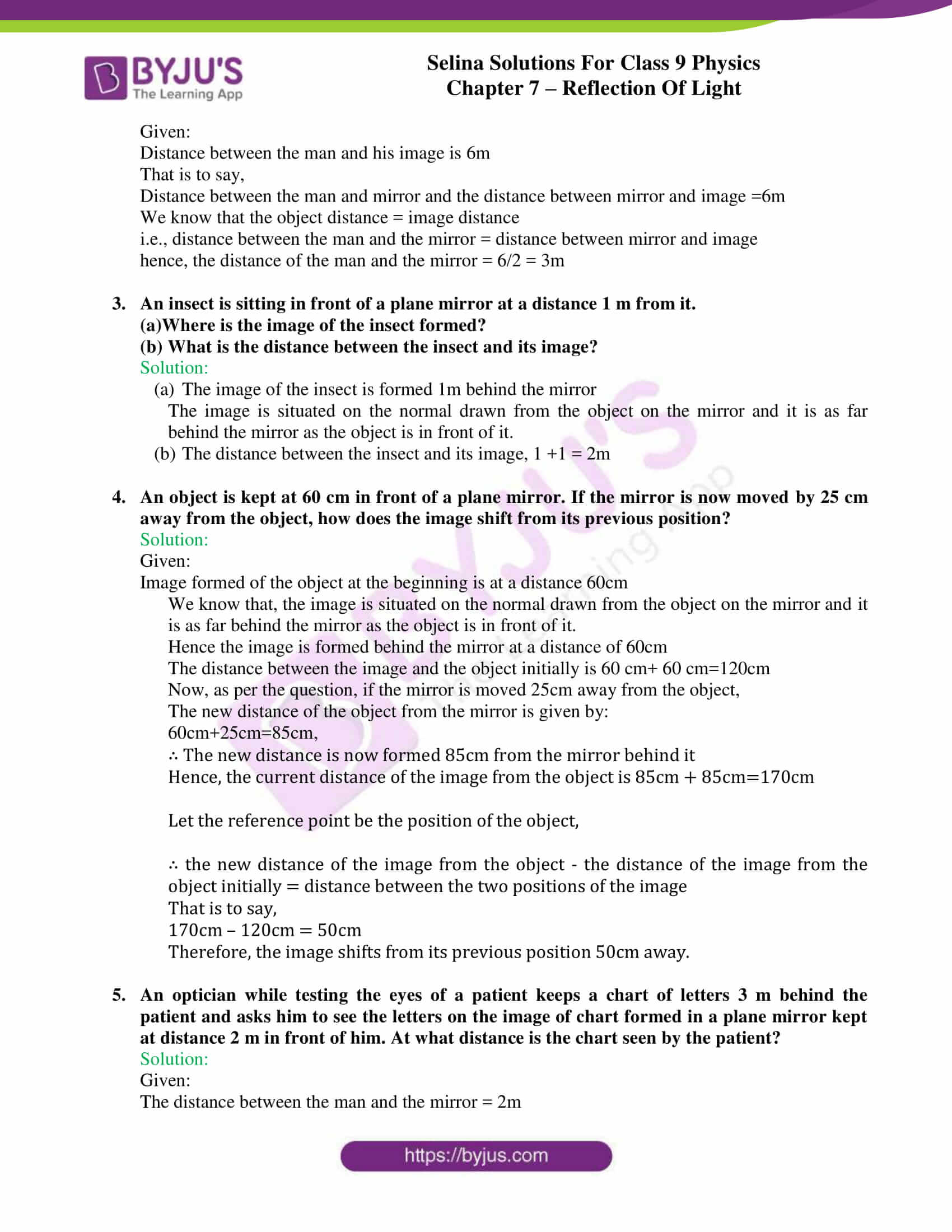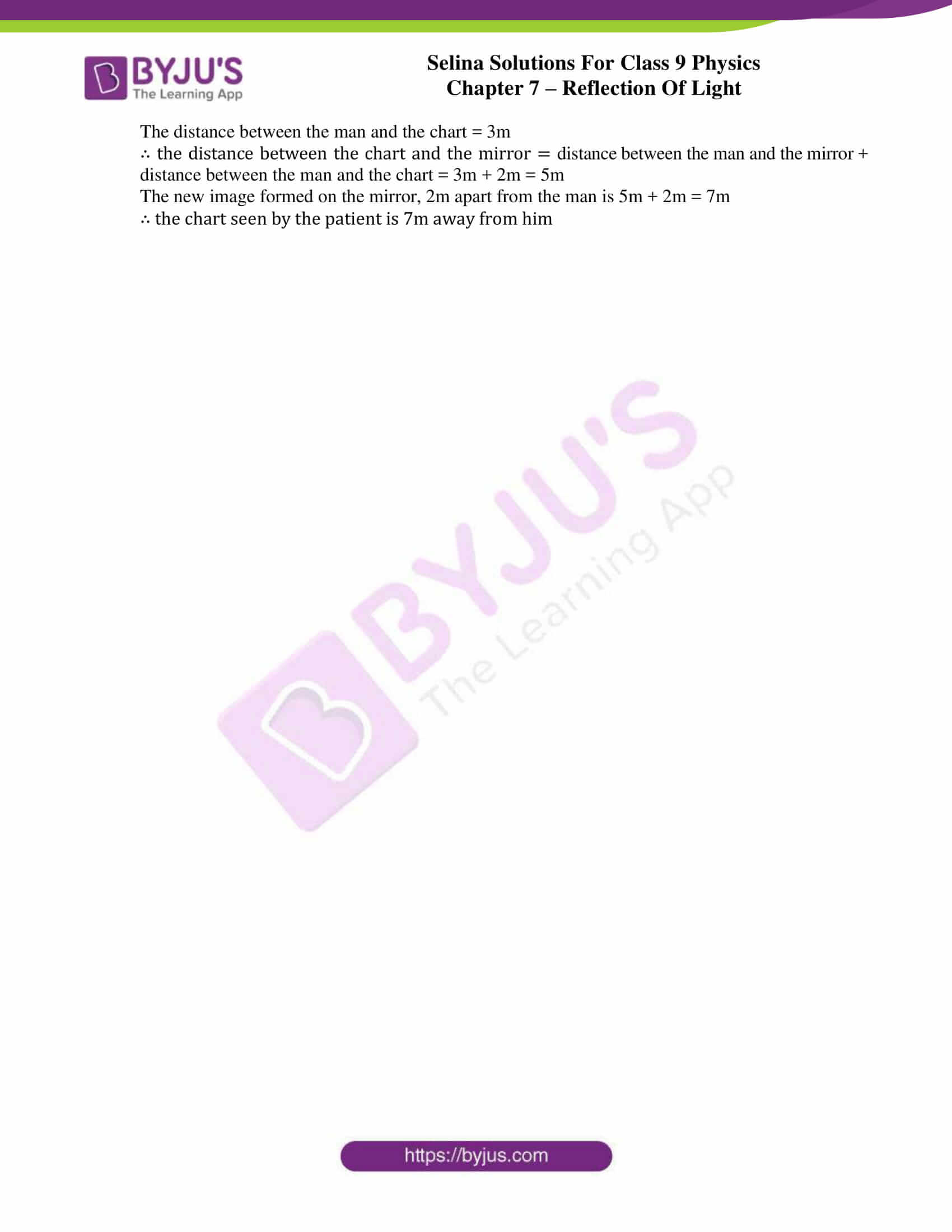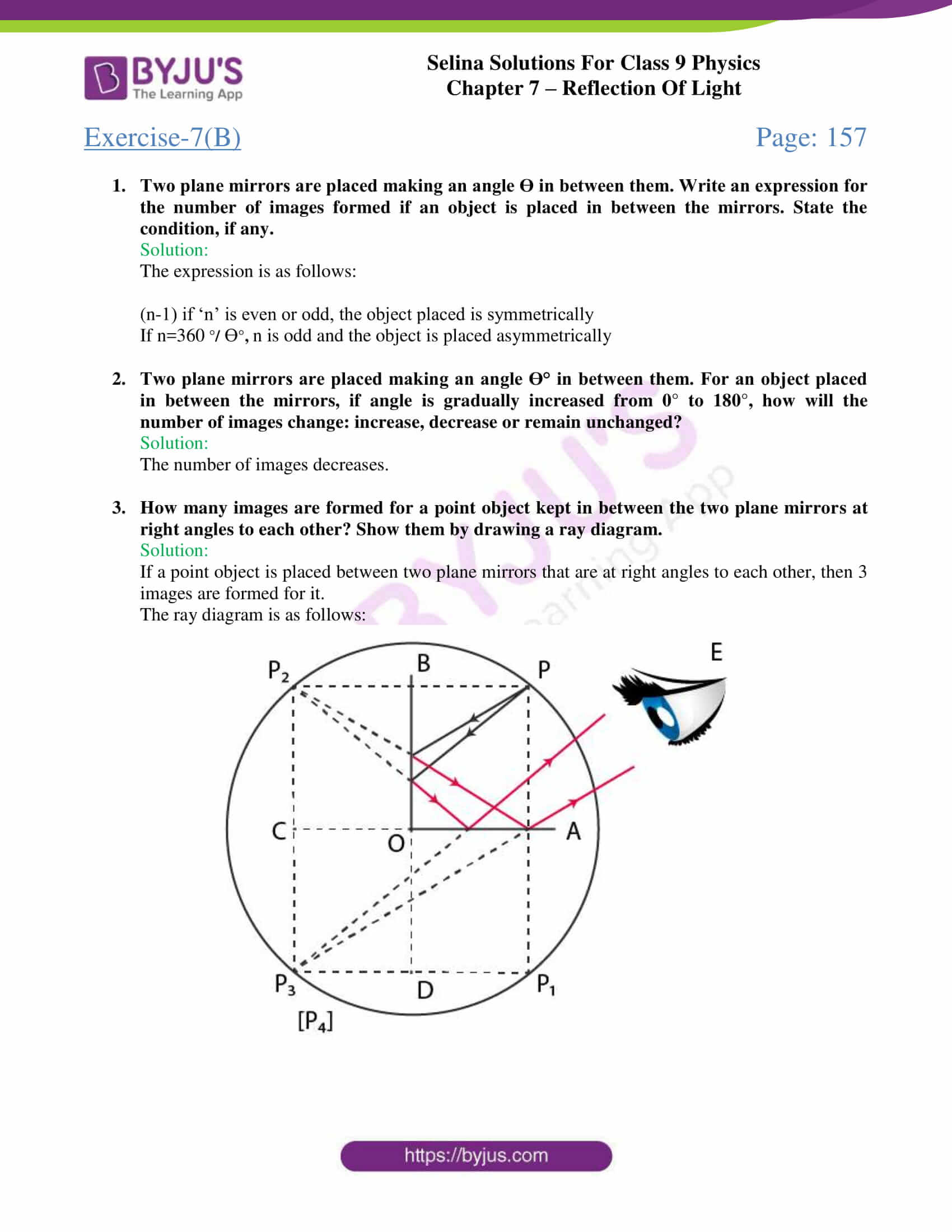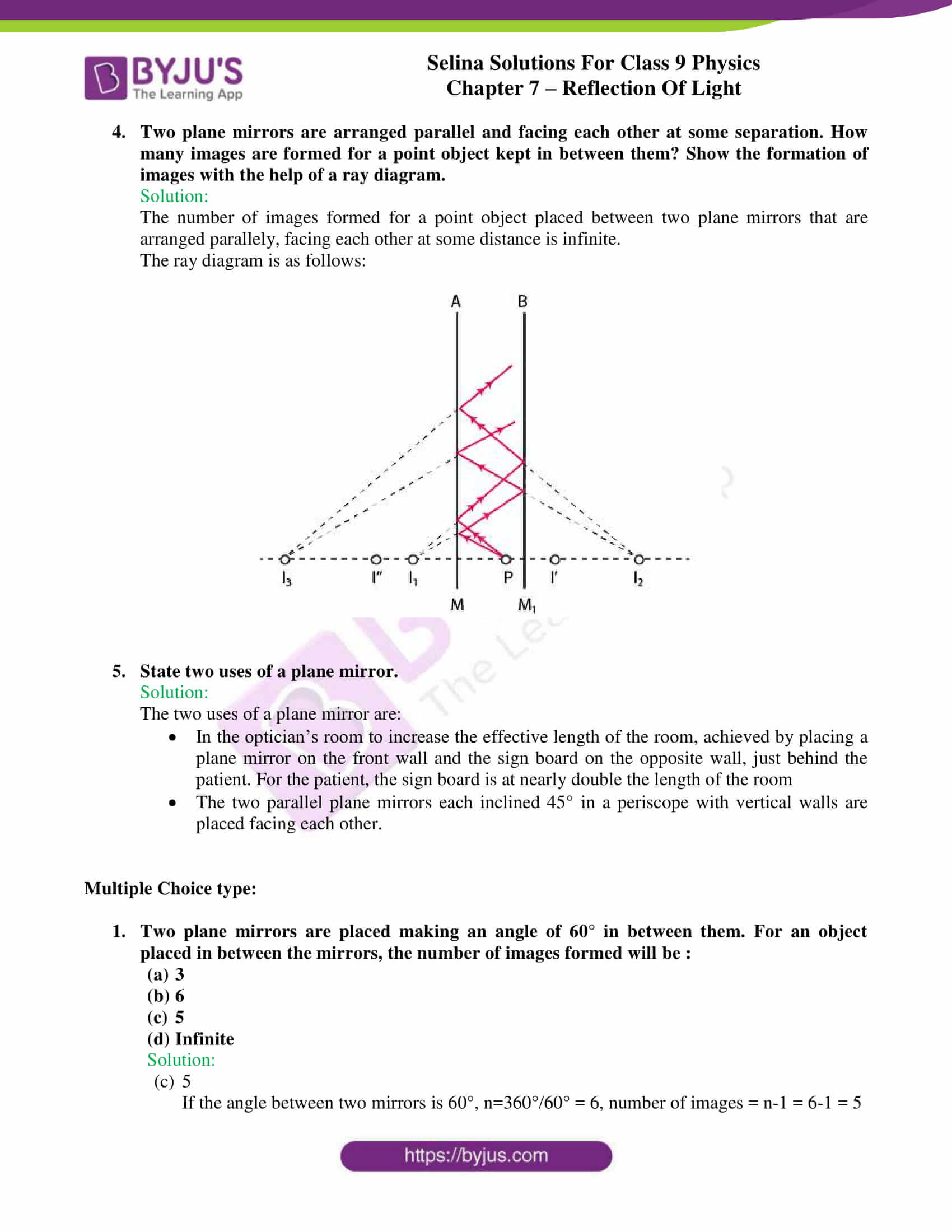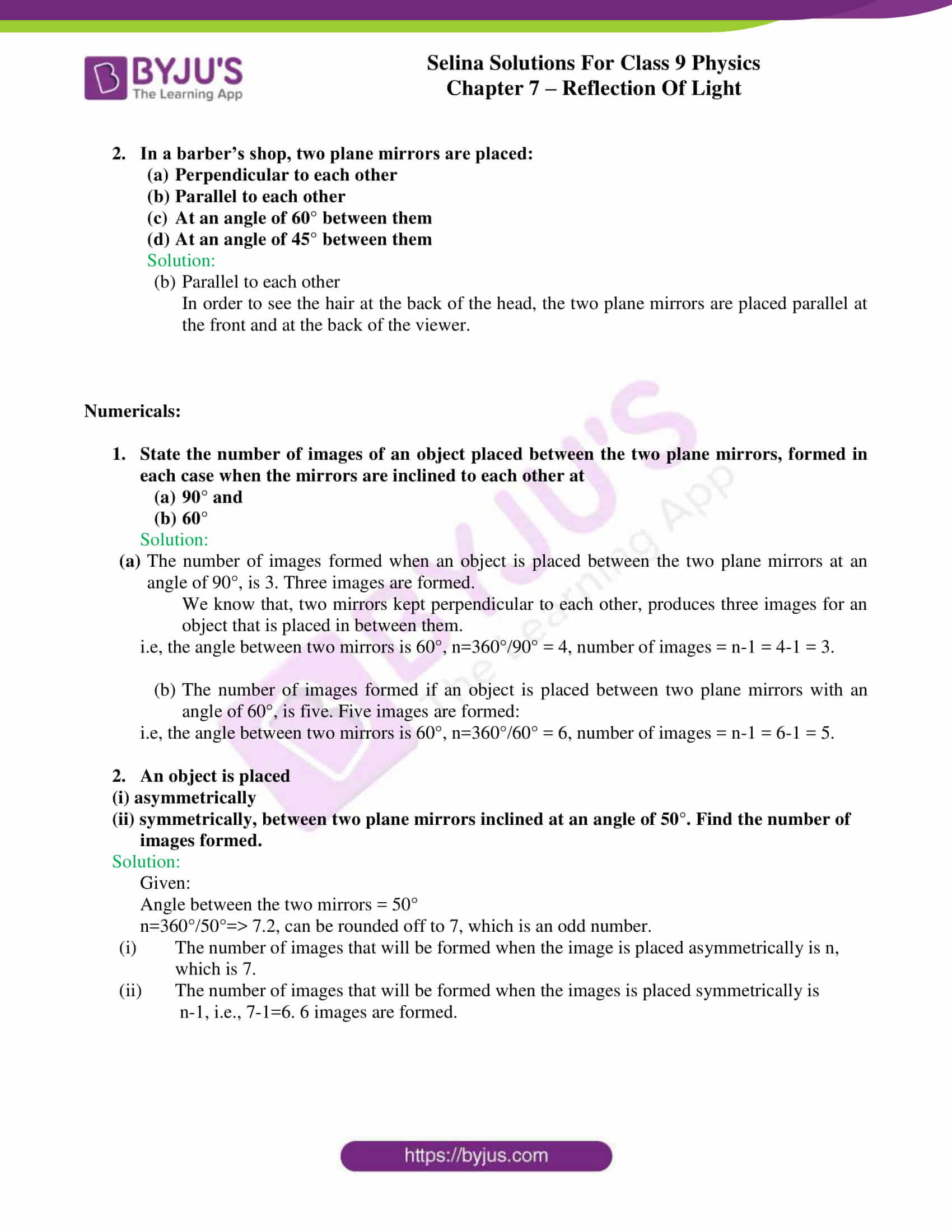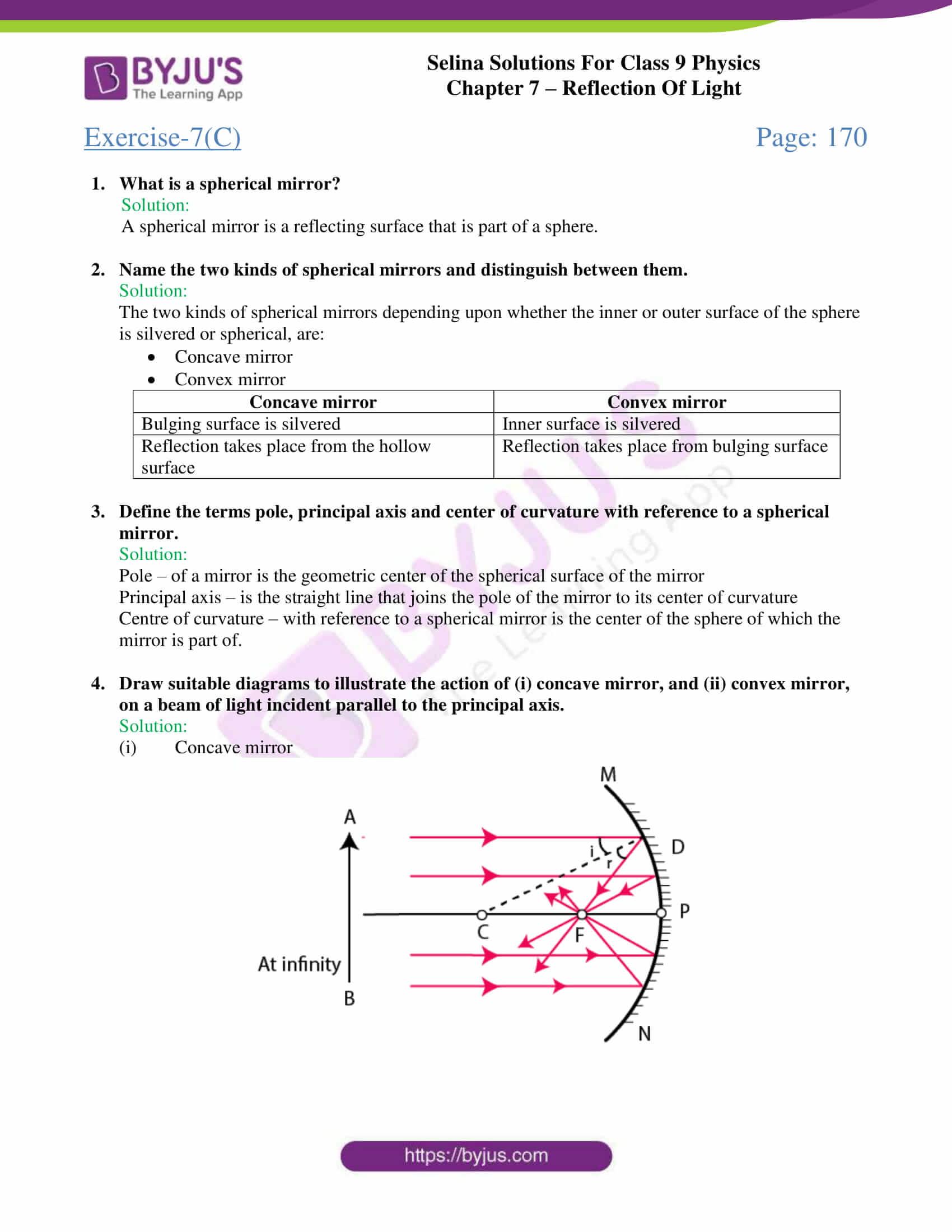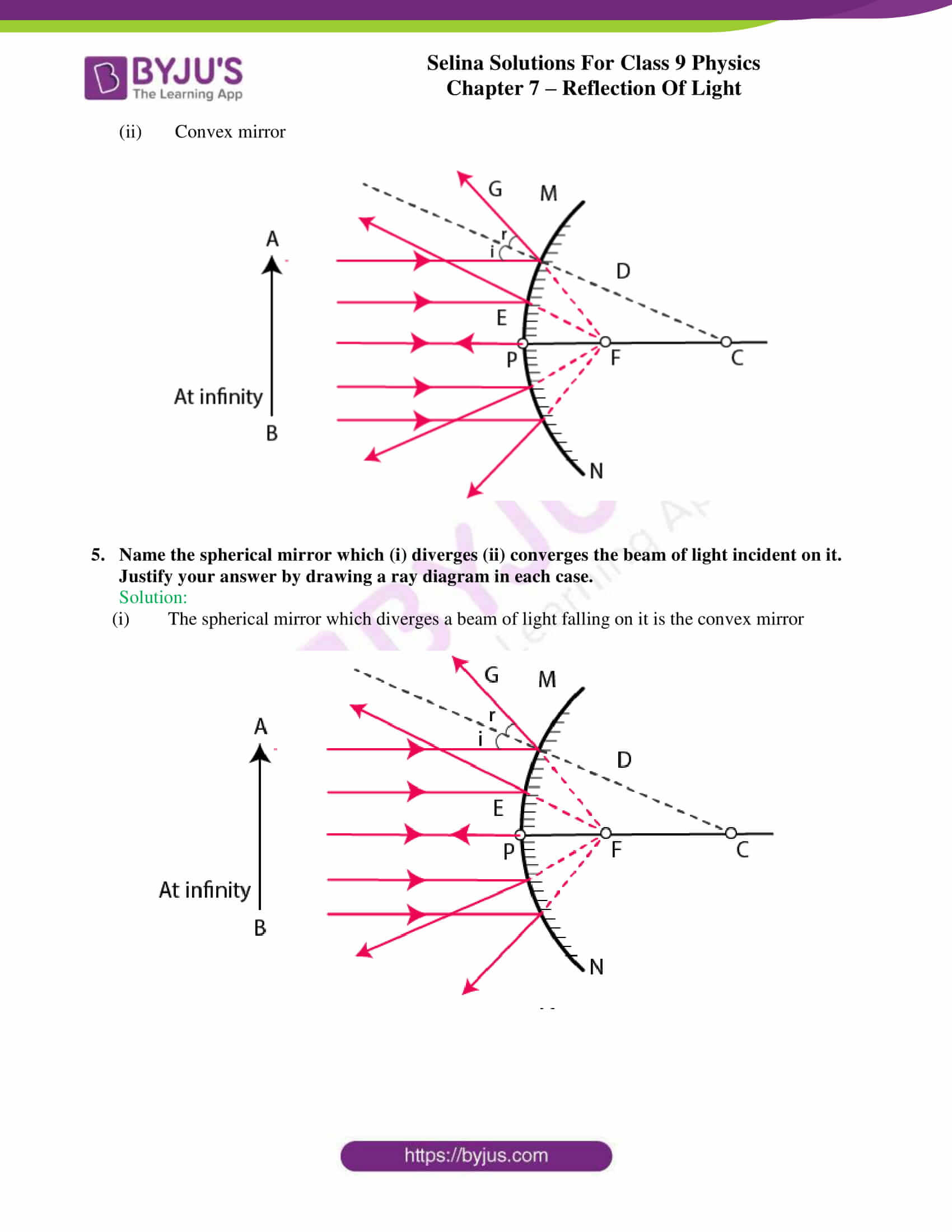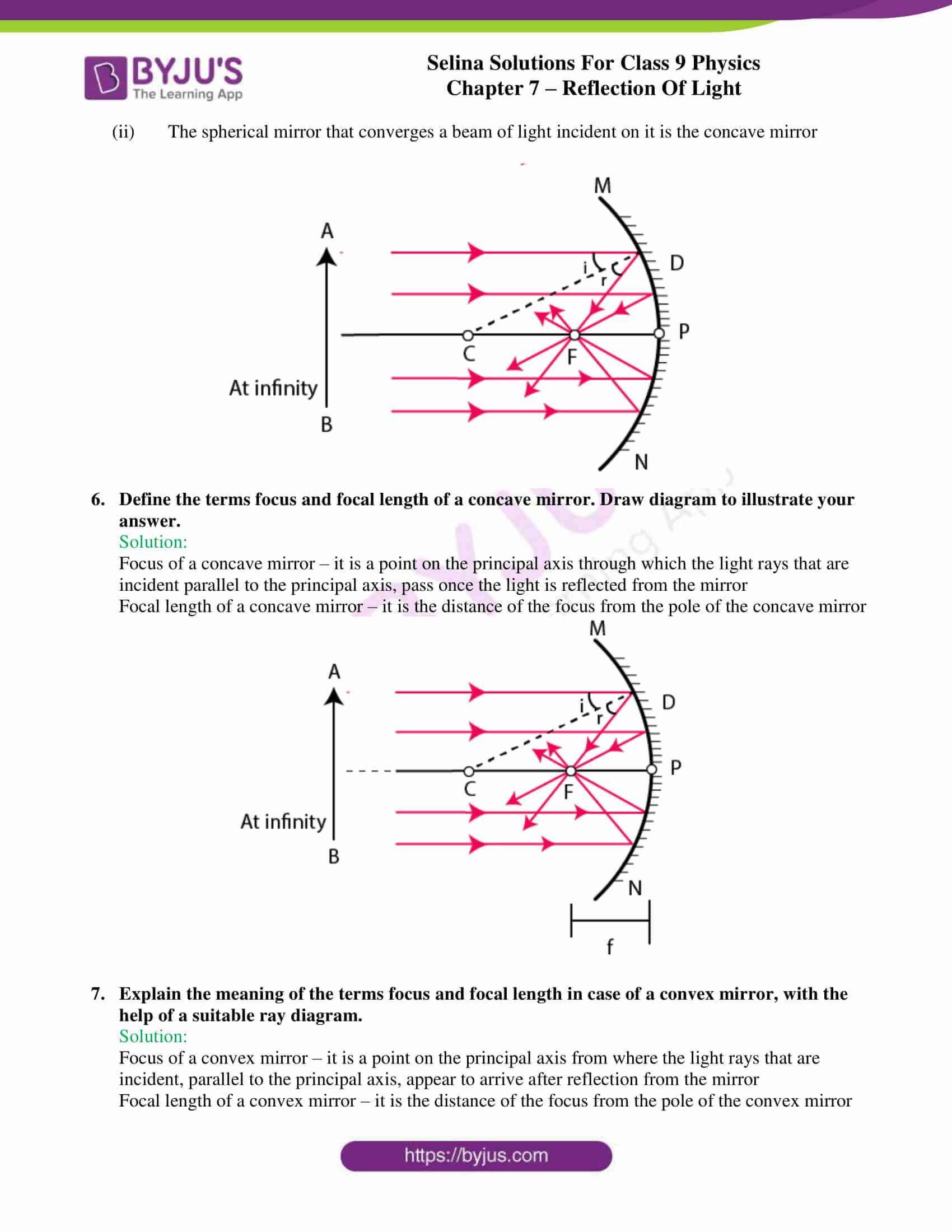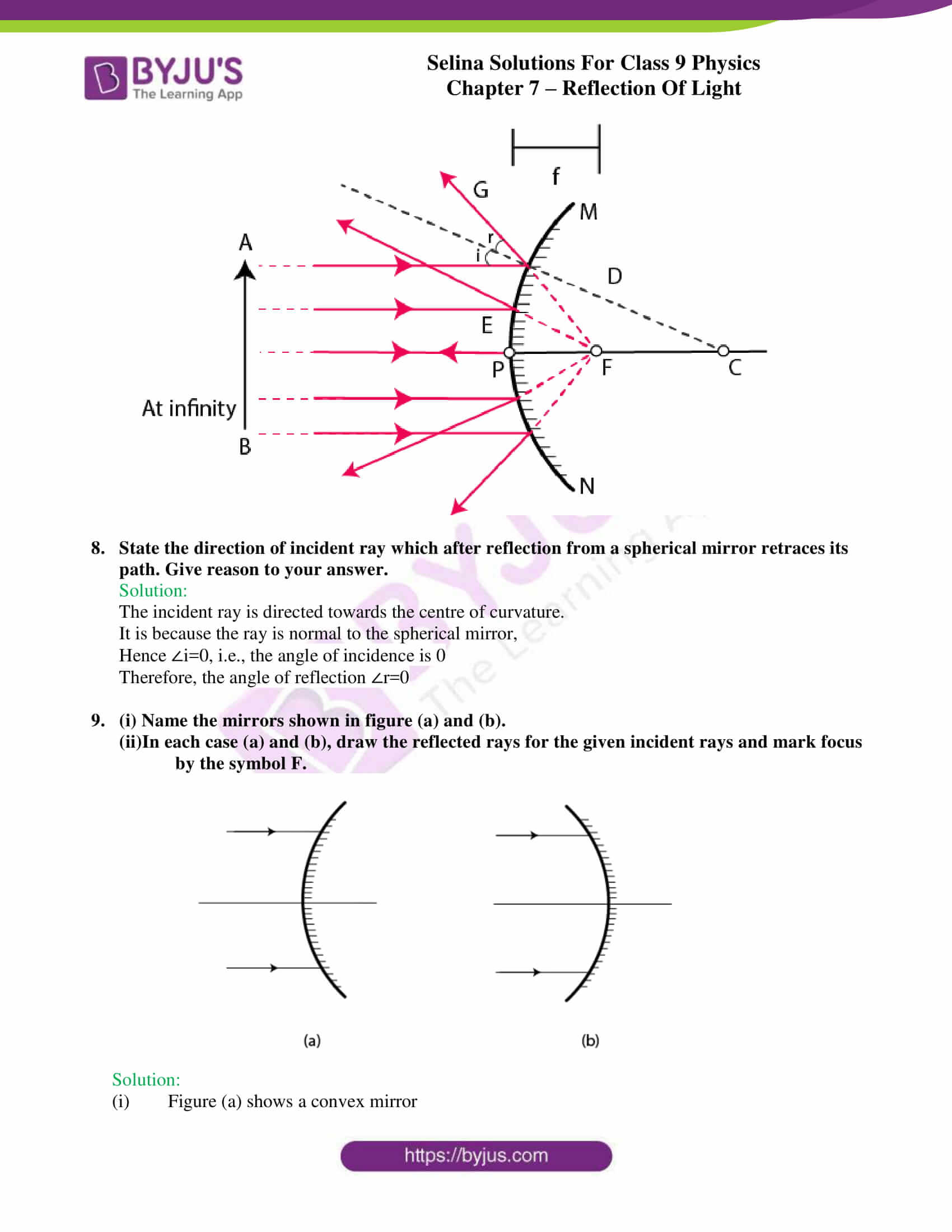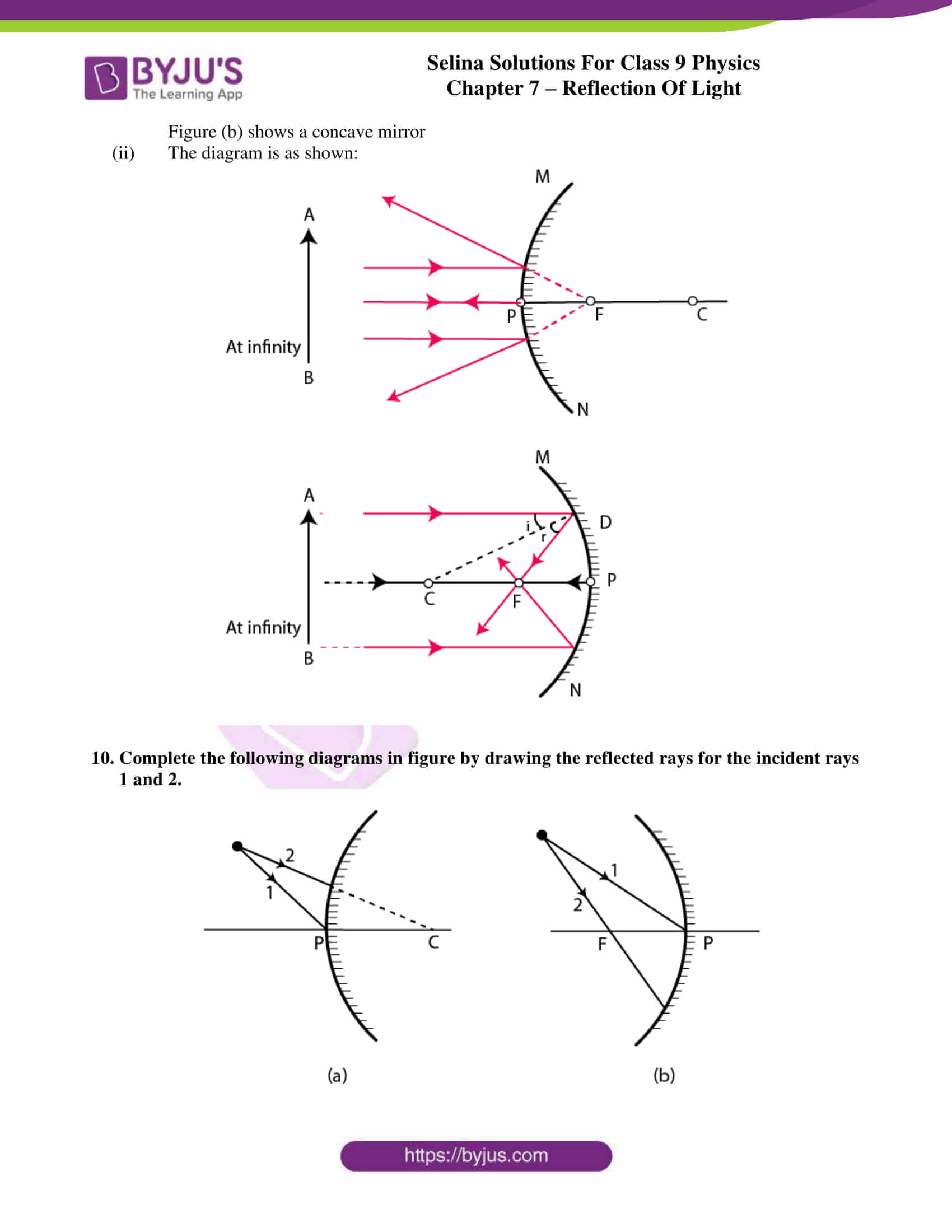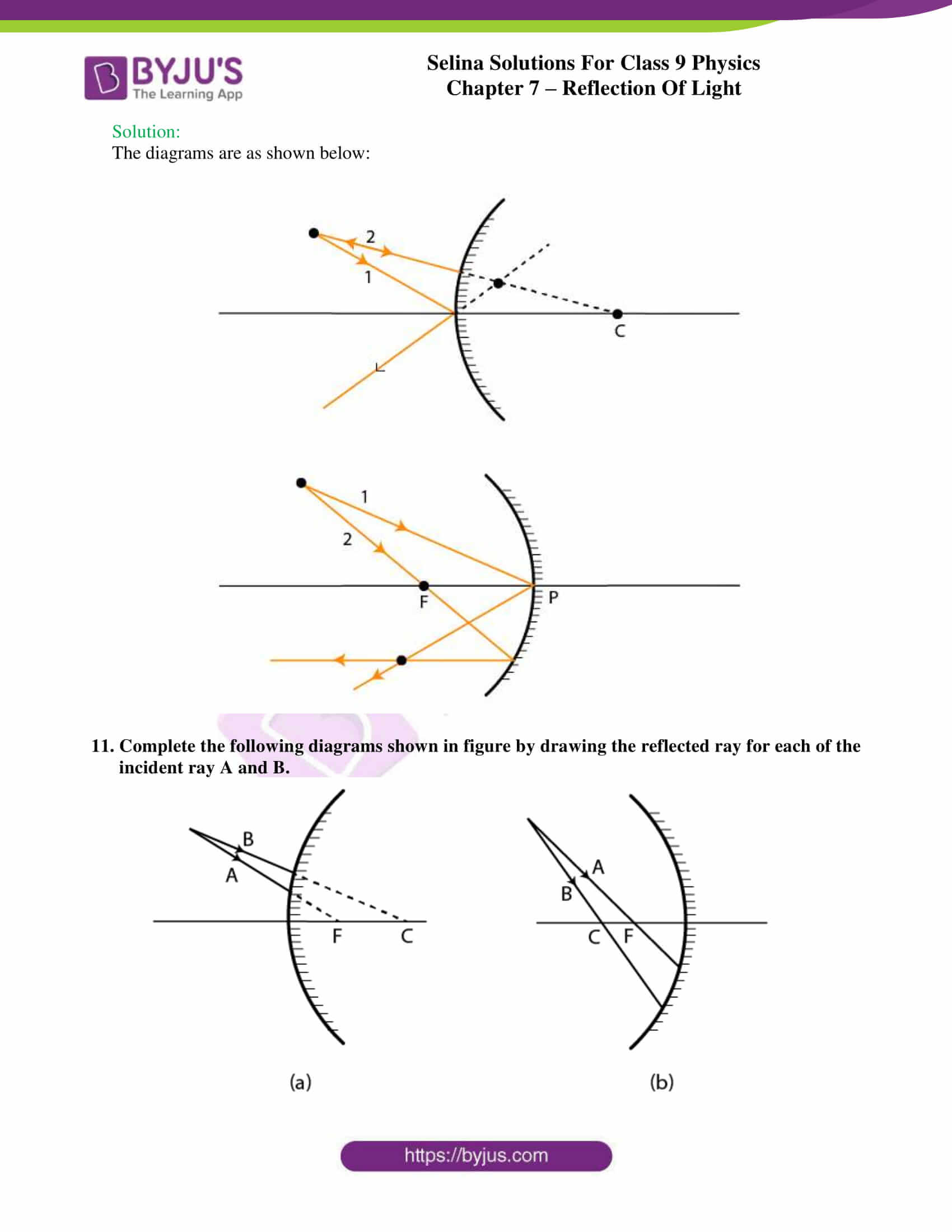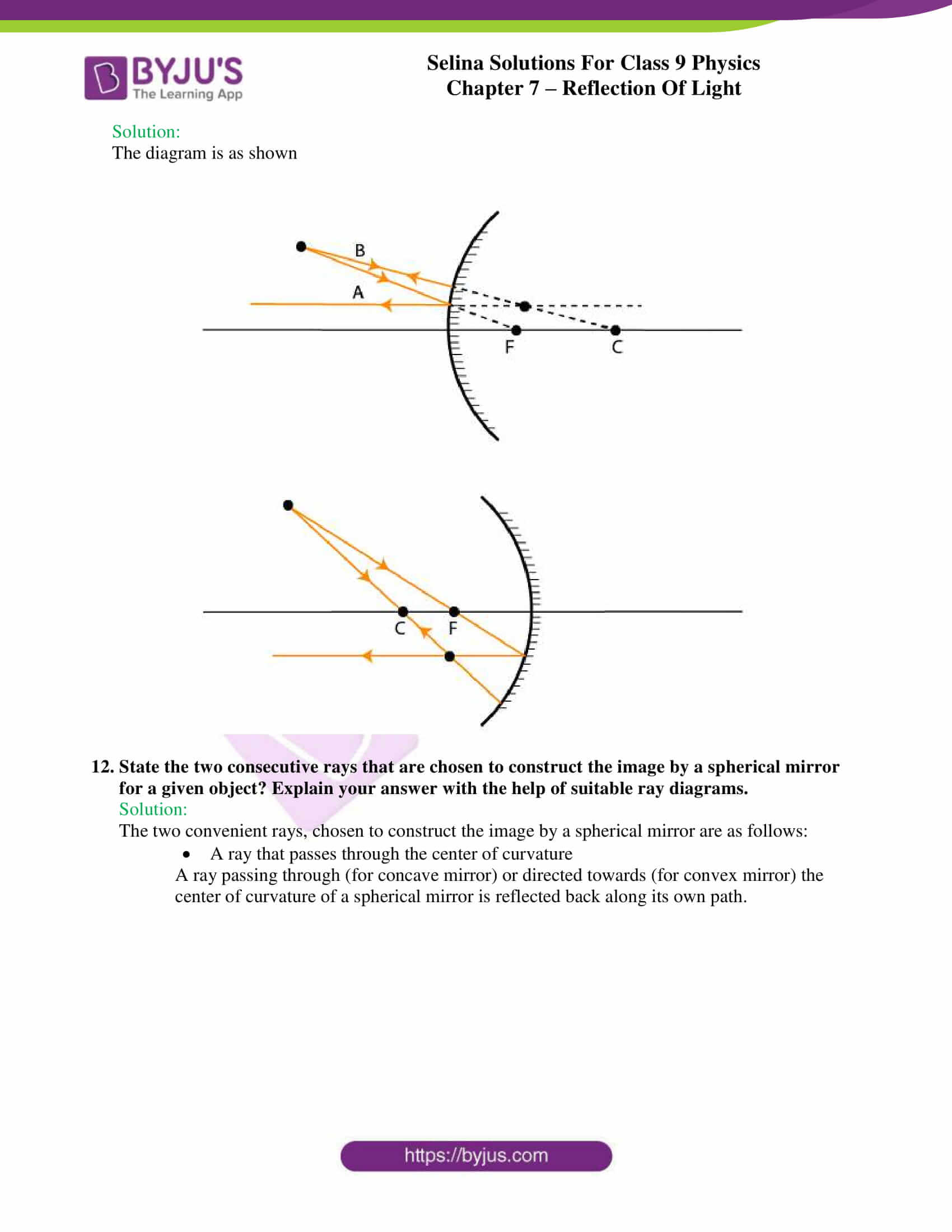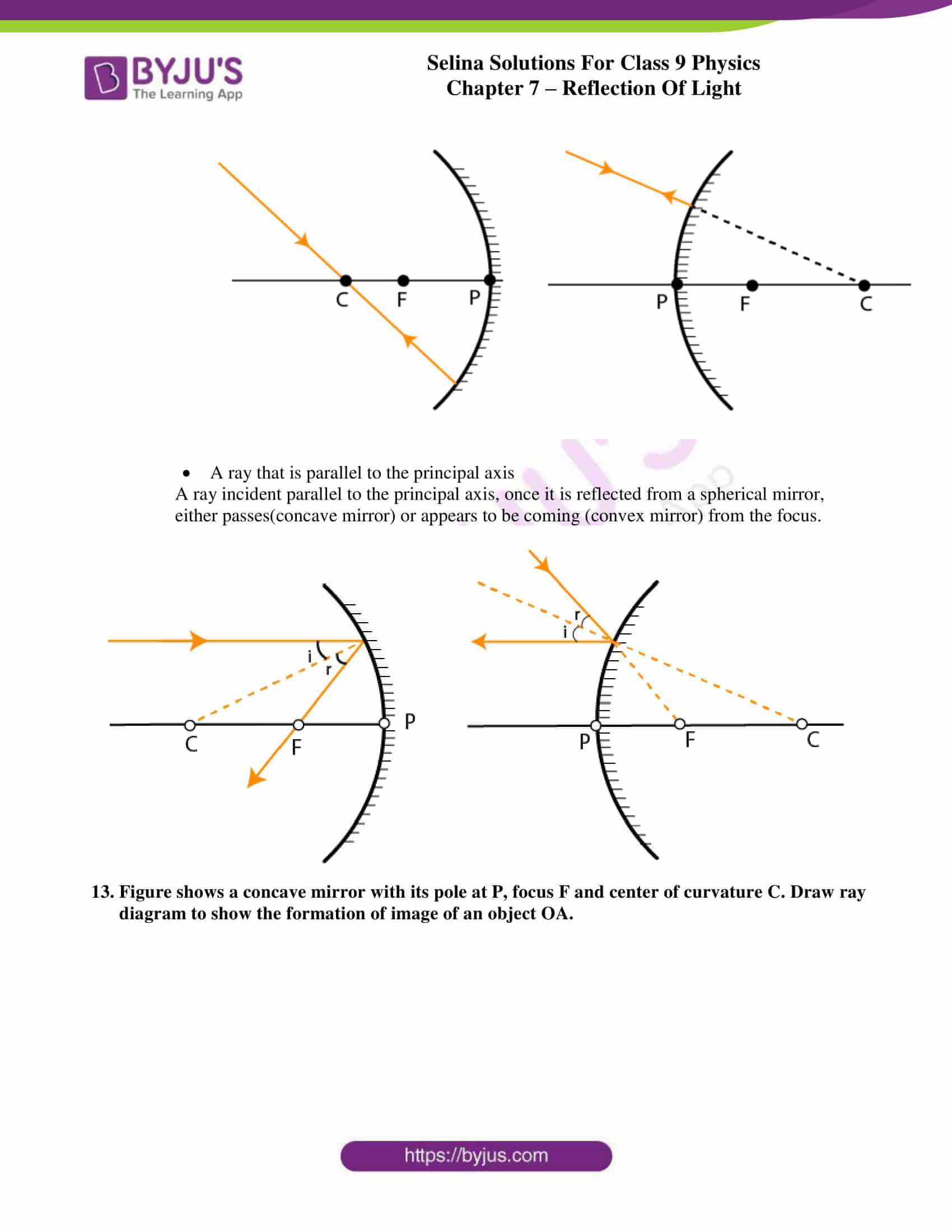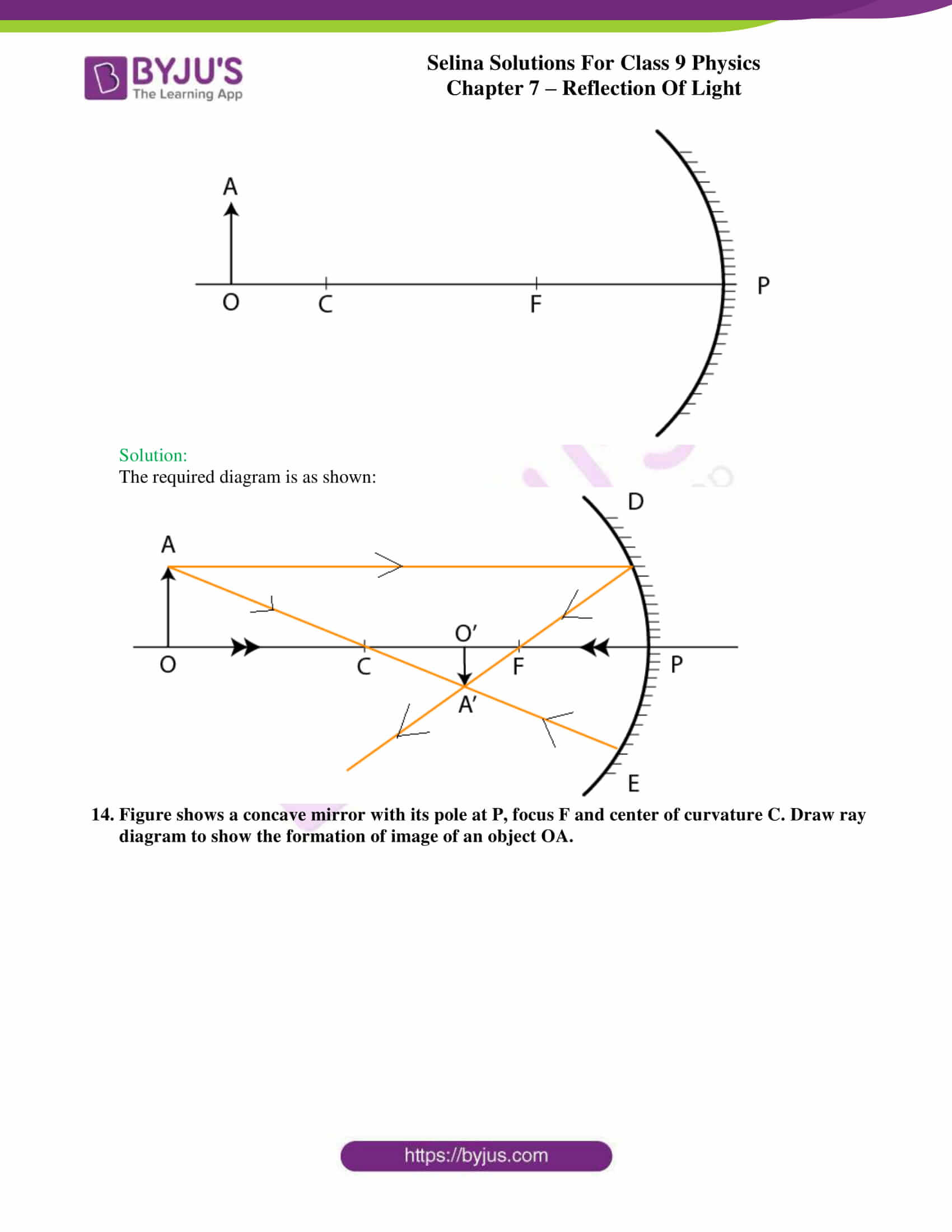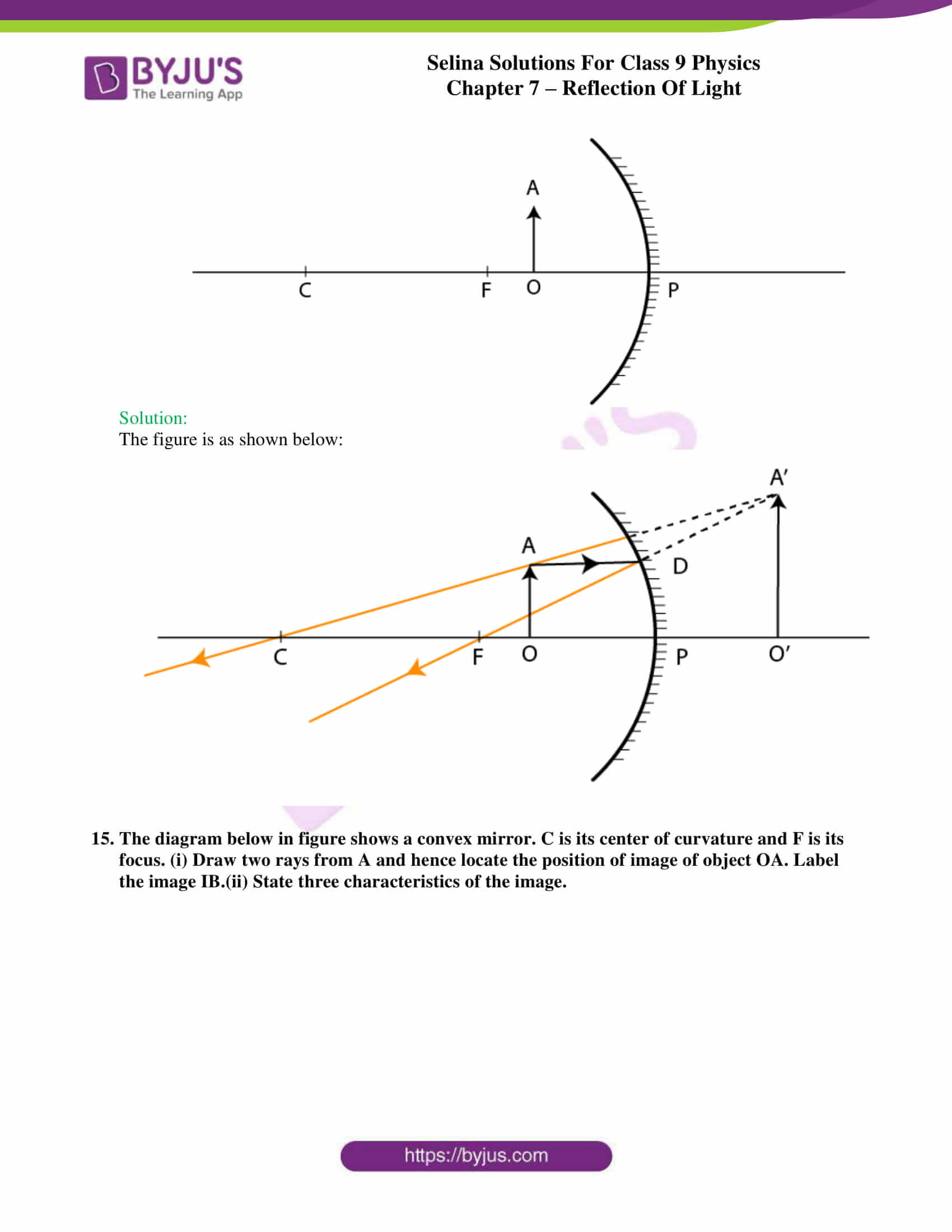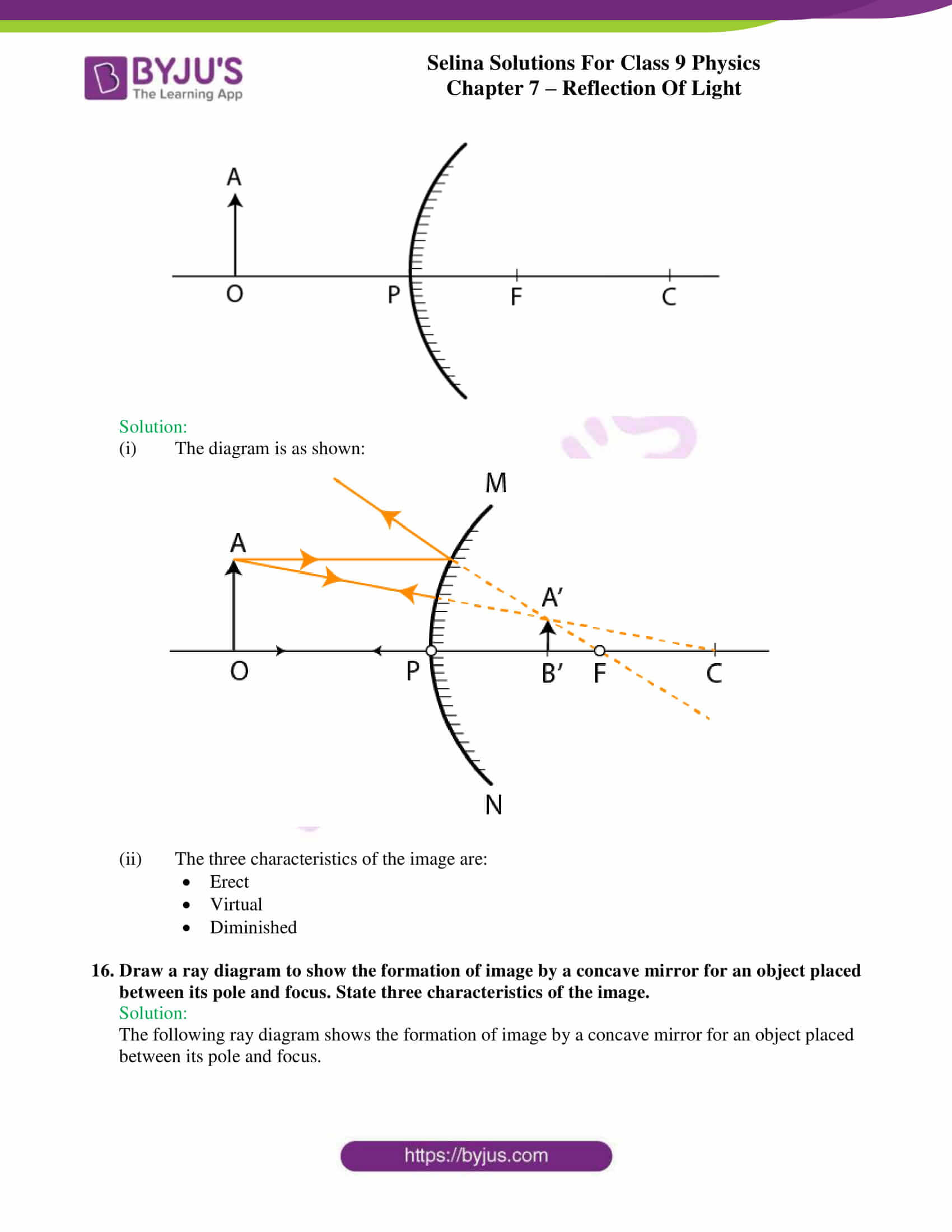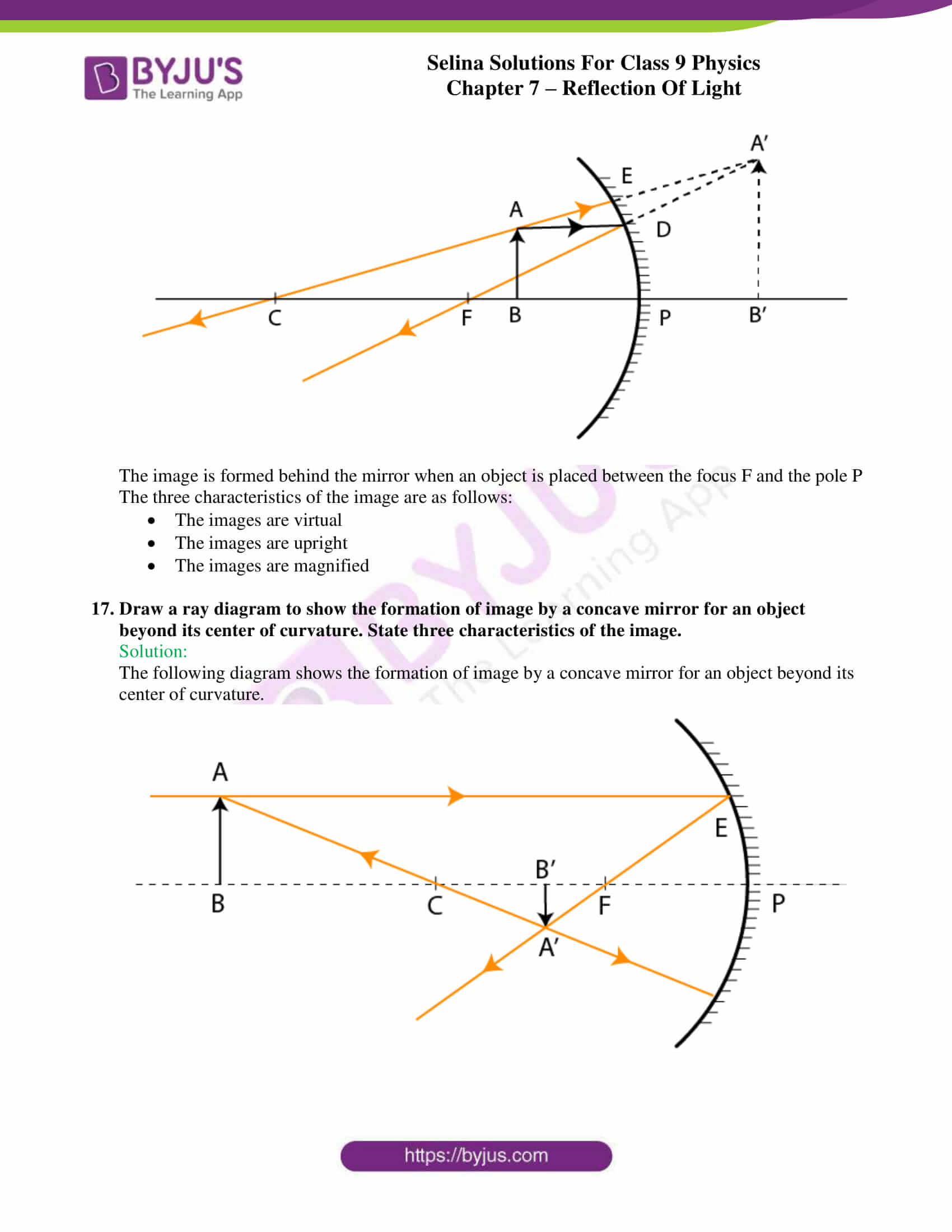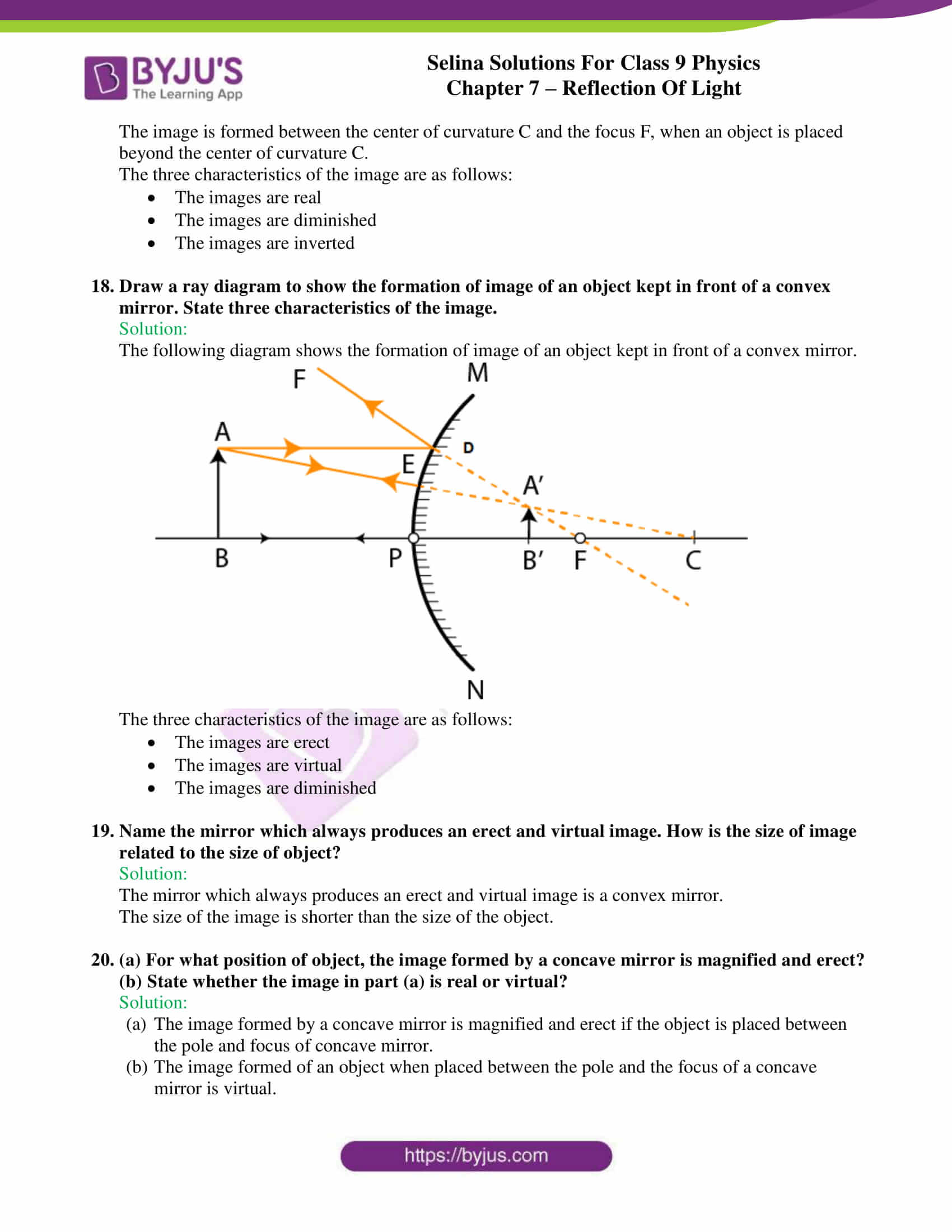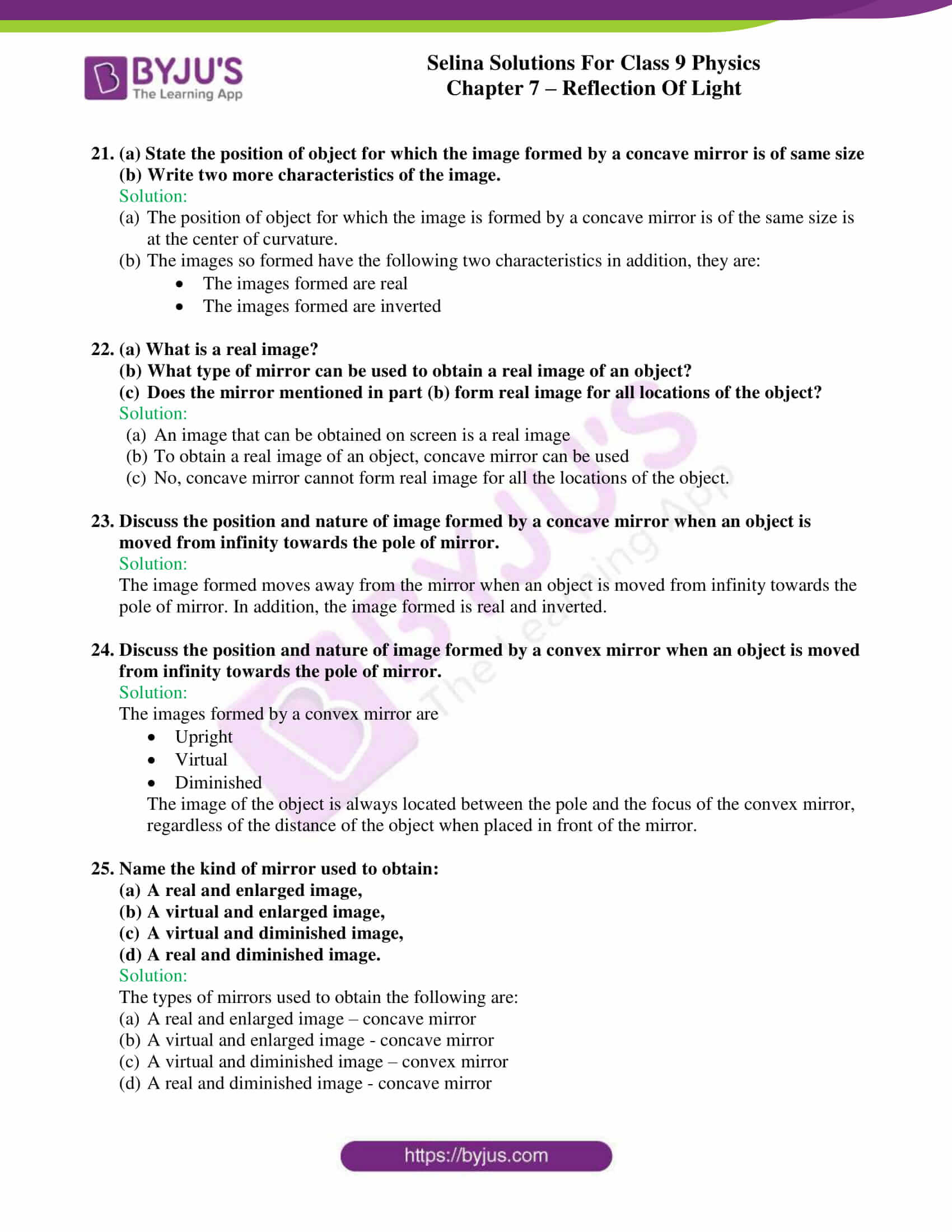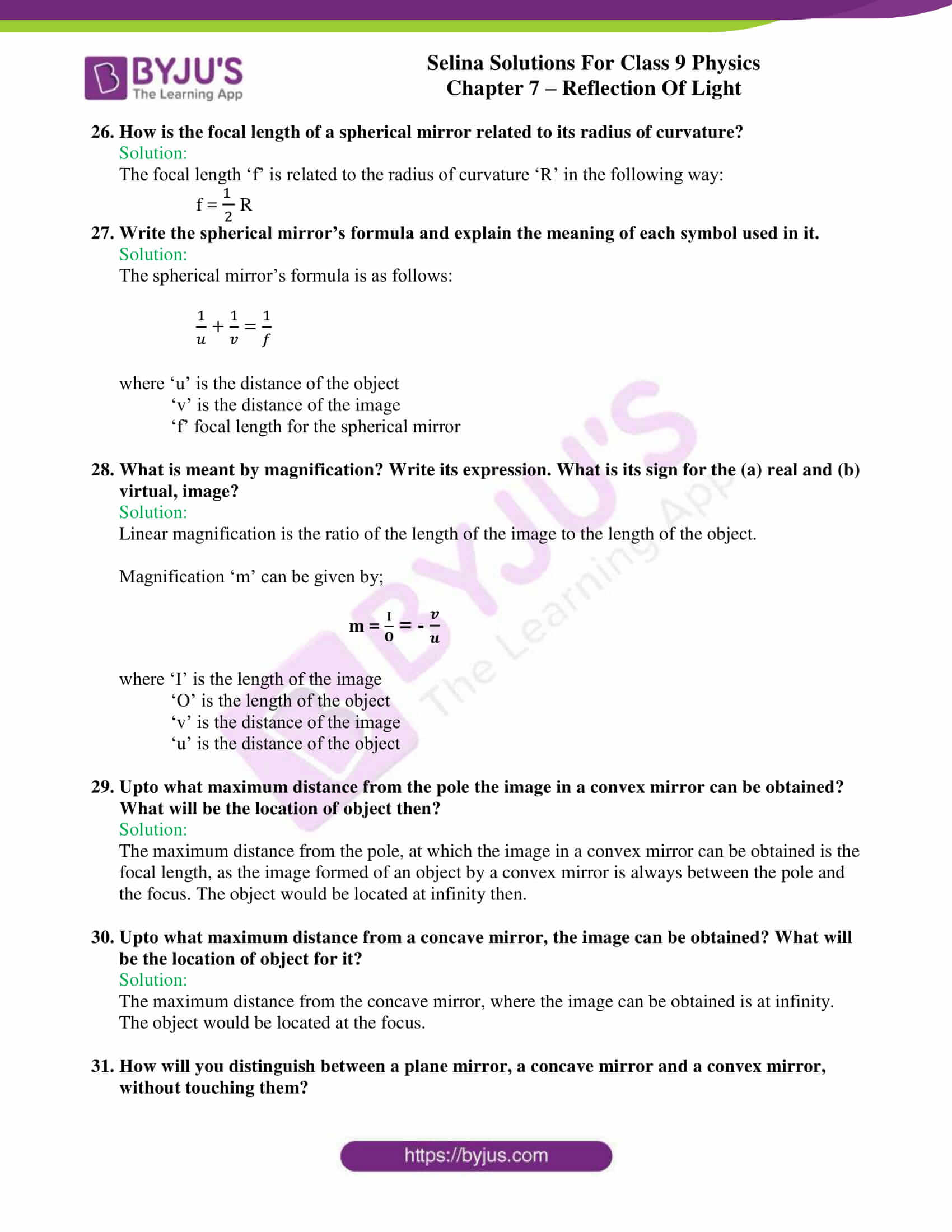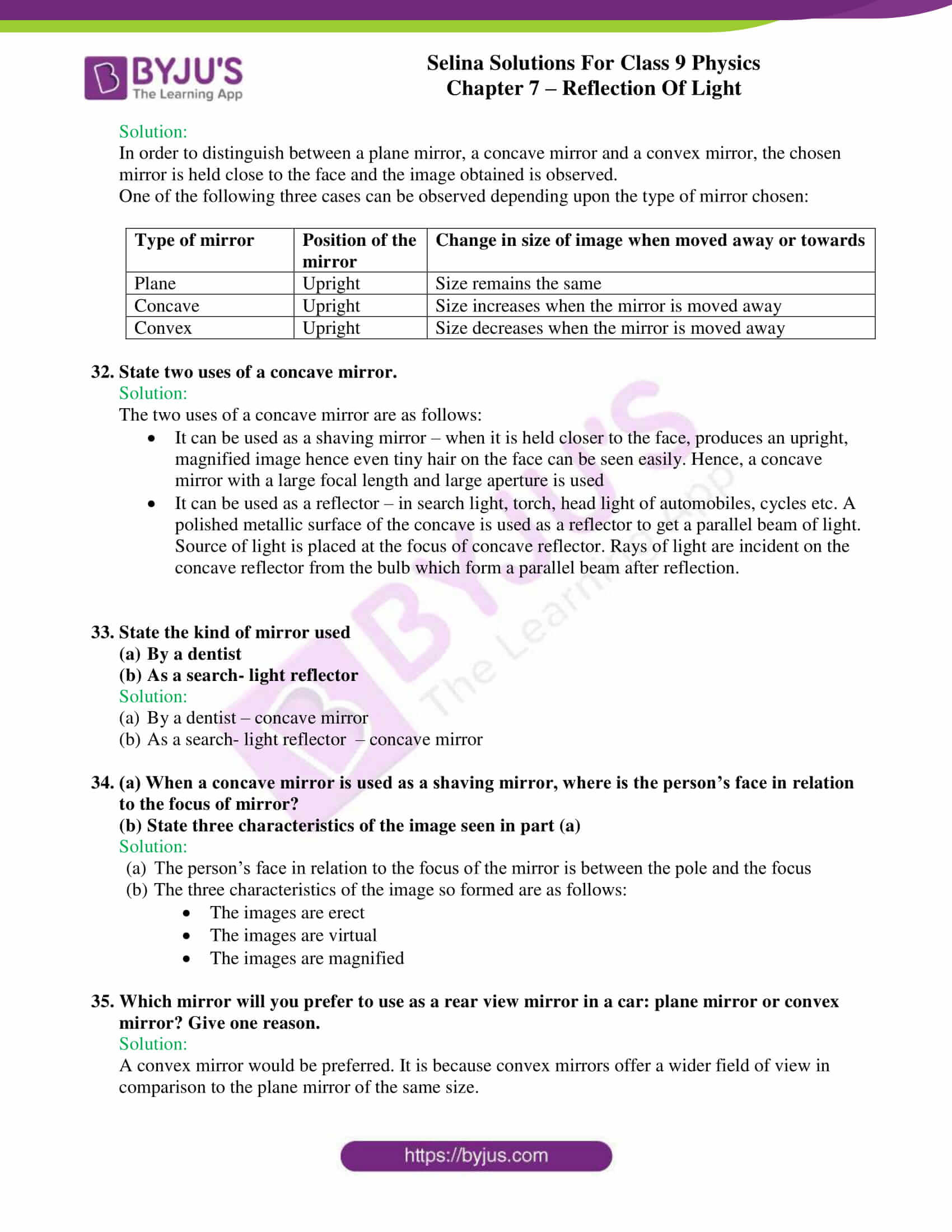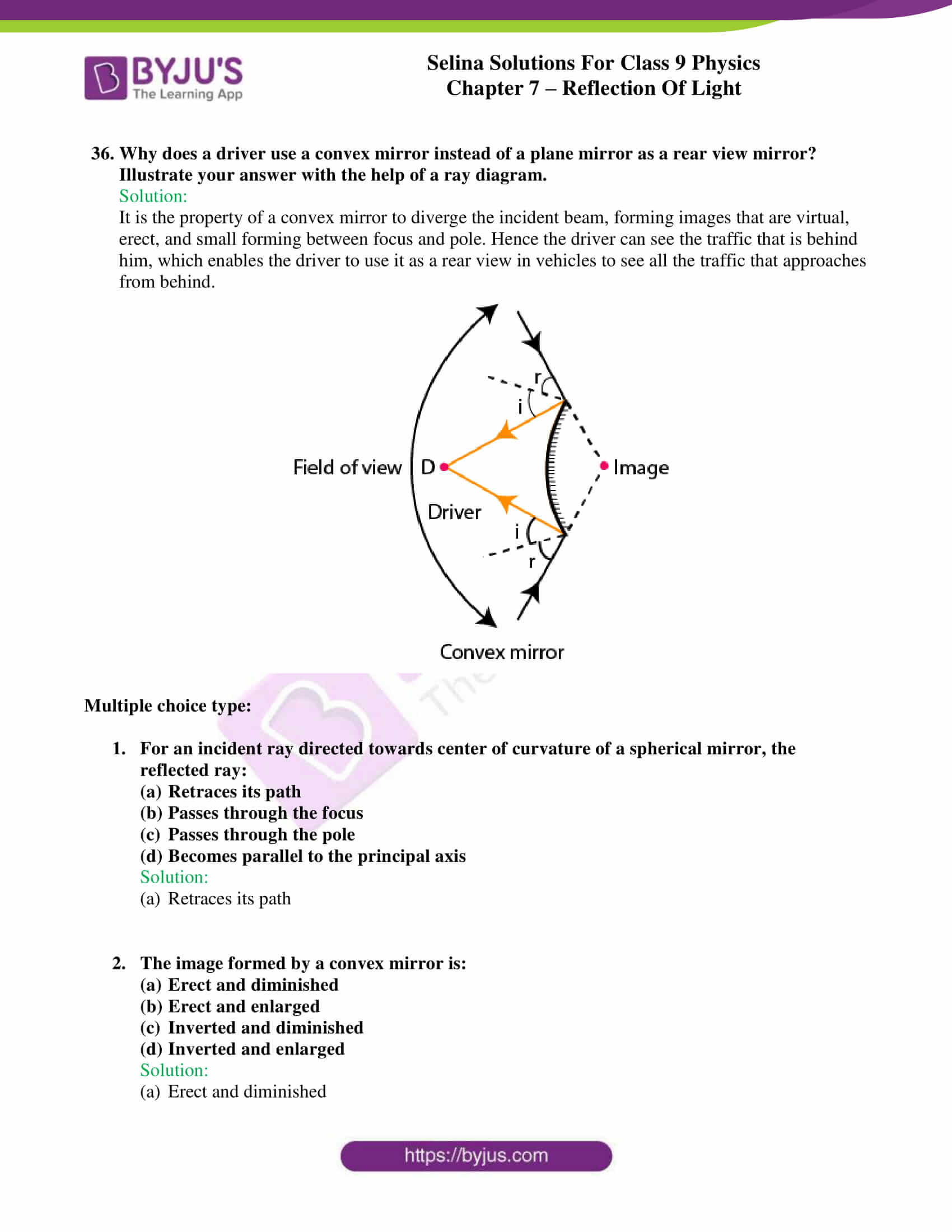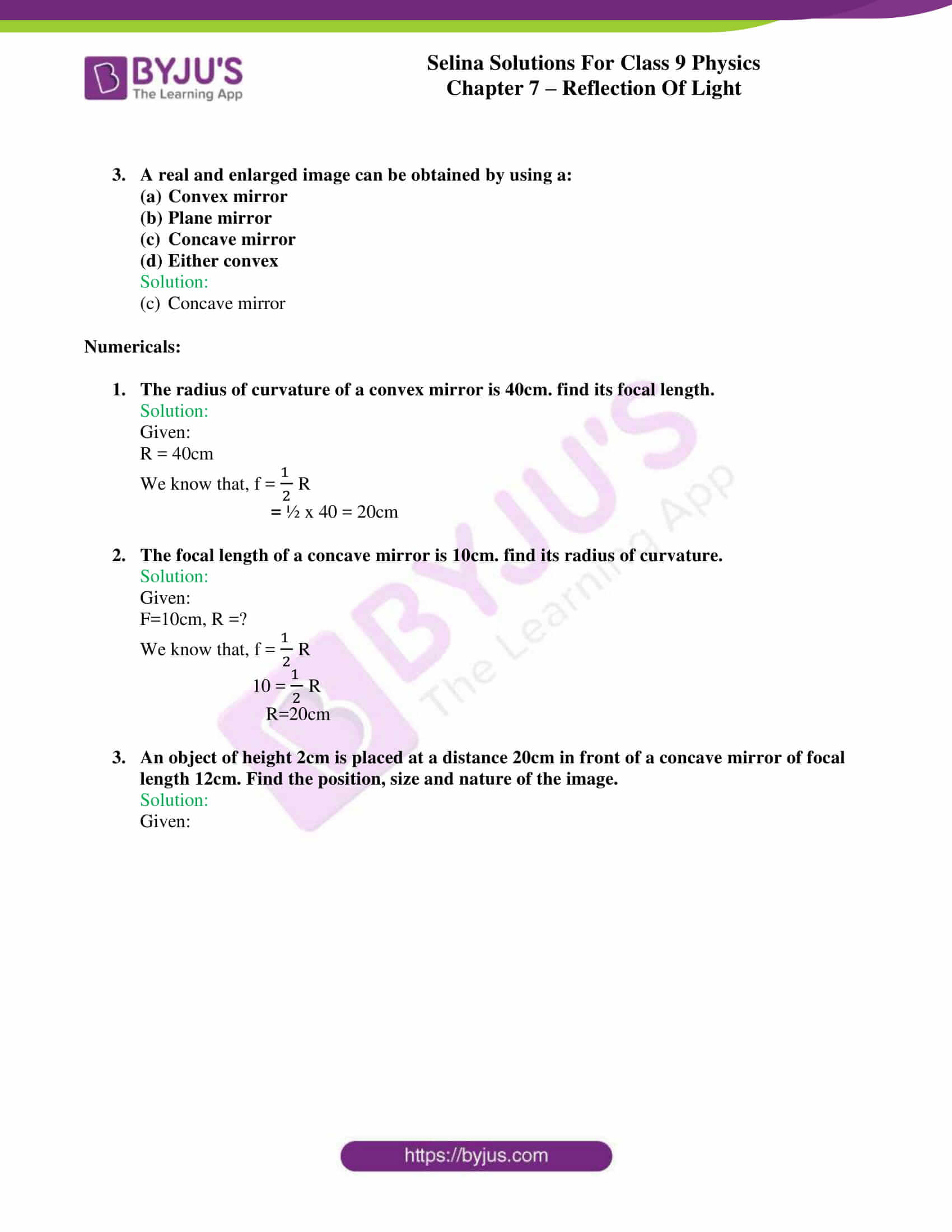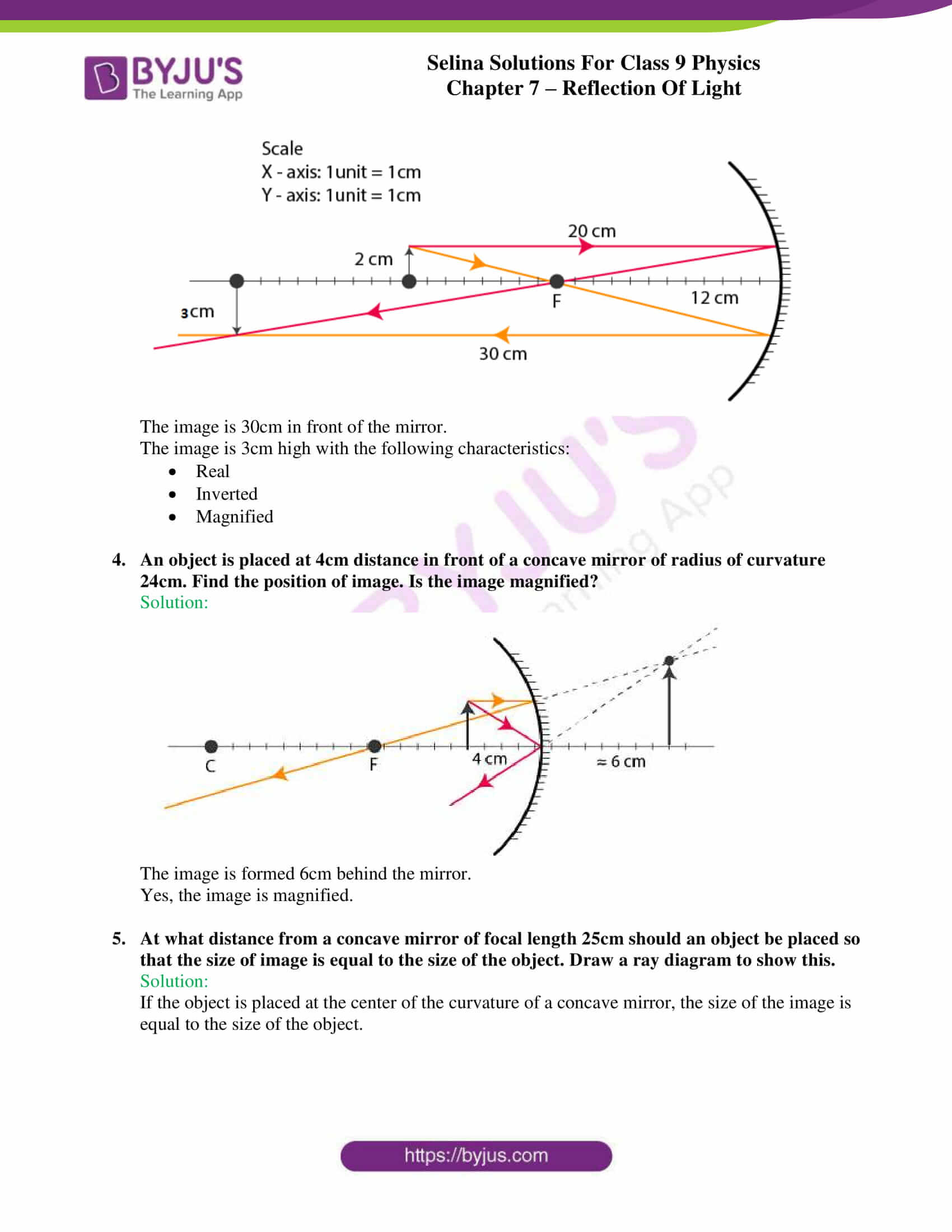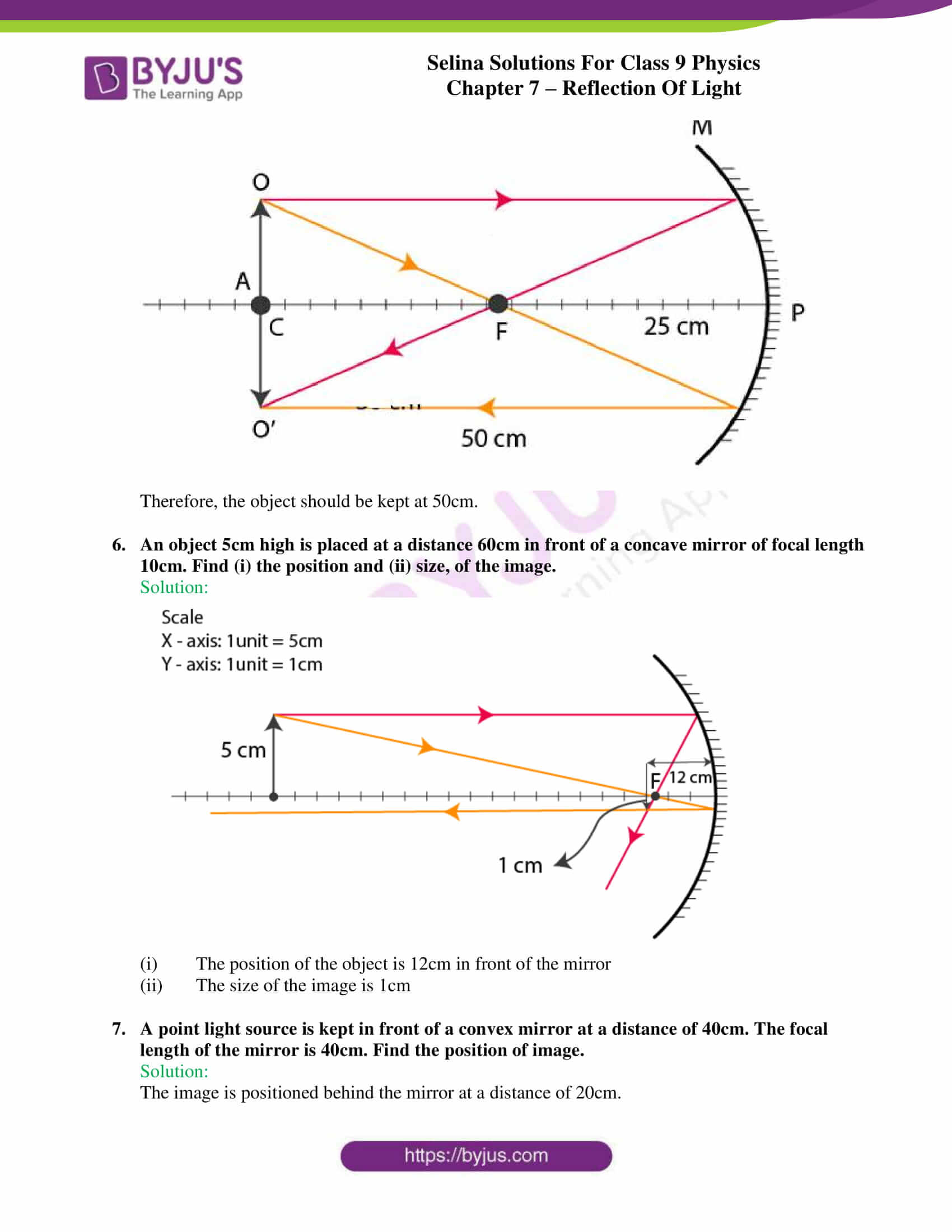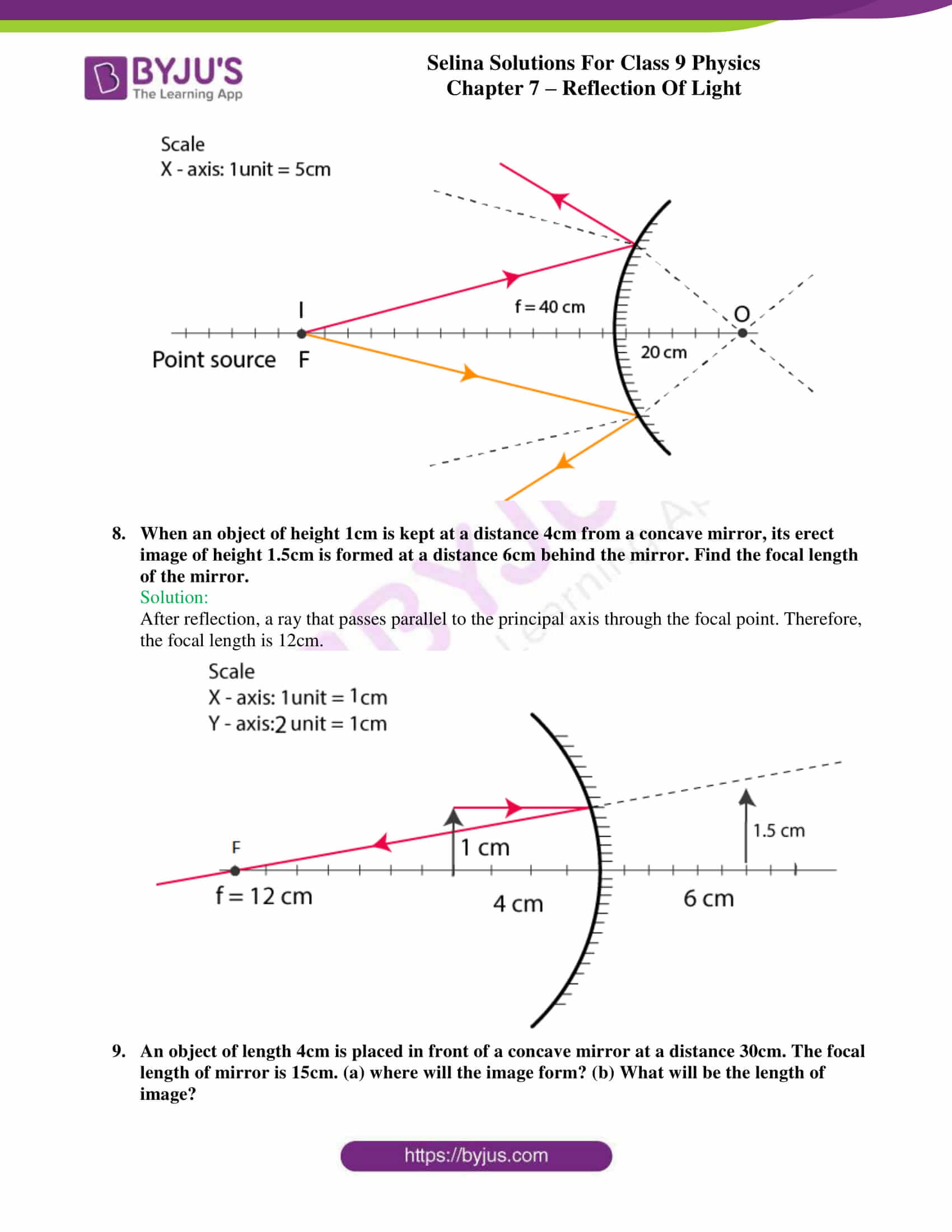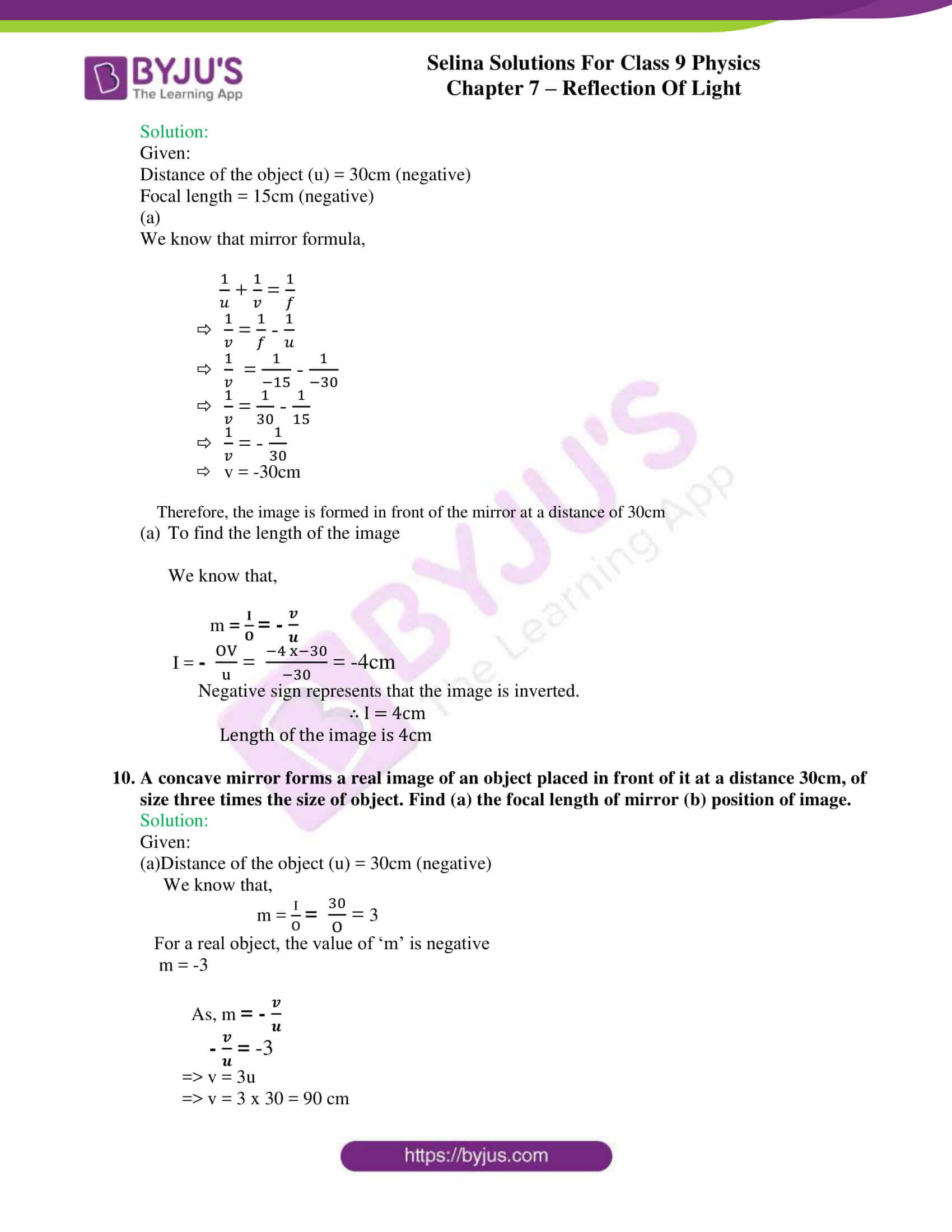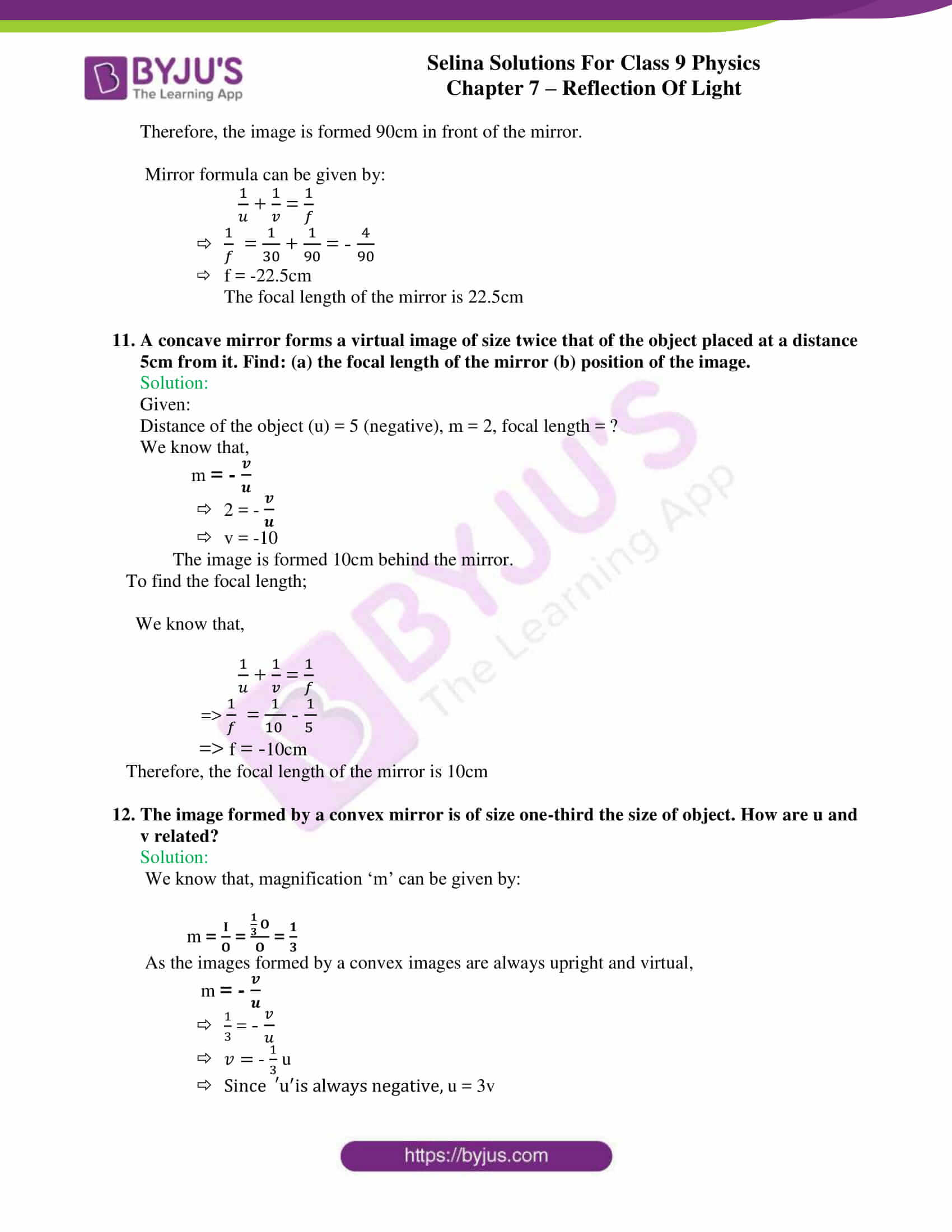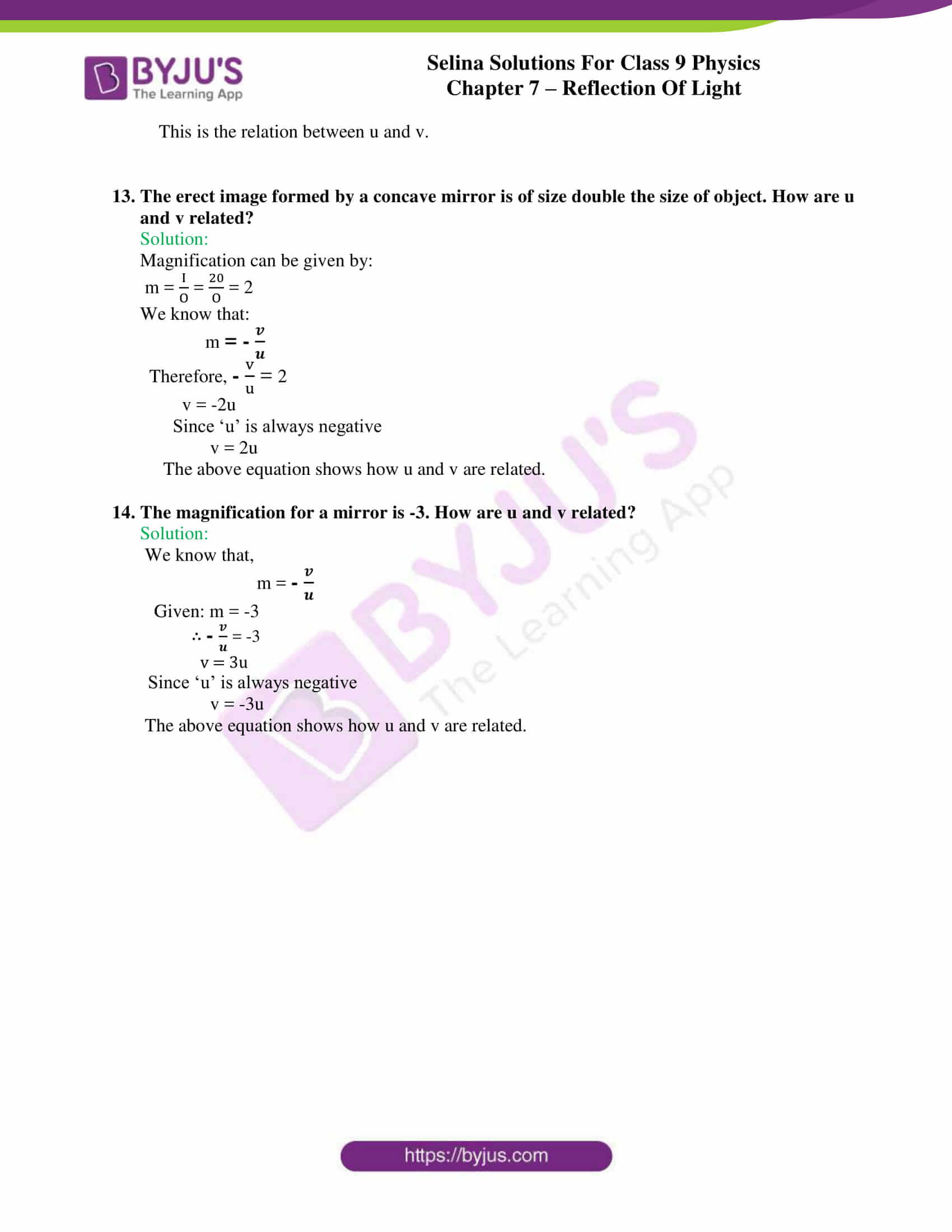## Selina Solutions for class 9 Physics Chapter 7 – Reflection of Light

ICSE Class 9 Physics 7 – Reflection of Light chapter is covered in the class 9 textbook under chapter 7. Light is electromagnetic radiation within a certain part of the electromagnetic spectrum. In the previous academic years, students have studied about the fundamentals, but in class 9, the concepts are taken one notch higher. The chapter discusses explains how light is reflected. When light returns into a medium after it has struck a surface, it is known as reflection. It is of different types(regular and irregular).

The chapter also explains laws of reflection, experimental verification for the laws of reflection, and the process of image formation through the process of reflection.

Overall, students understand how light is reflected, how images are formed, the different factors governing the process, the various terminologies involved, ray diagrams, differences between mirrors along with suitable examples.

List of subtopics covered in Chapter 7 – Reflection of Light

 Number Subtopic 7.1 Reflection of light 7.2 Some terms related with reflection 7.3 Laws of reflection 7.4 Experimental verification of the laws of reflection 7.5 Formation of image by reflection 7.6 Image of a point object formed by a plane mirror 7.7 Image of an extended object formed by a plane mirror 7.8 Position of image 7.9 Lateral inversion 7.10 Characteristics of the image formed by a plane mirror 7.11 Images formed in two inclined mirrors 7.12 Images formed in a pair of mirrors placed parallel to each other 7.13 Images formed by two mirrors placed perpendicular to each other 7.14 Uses of plane mirror 7.15 Spherical mirror 7.16 Brief introduction of terms related to a spherical mirror 7.17 Reflection of a light ray from a spherical mirror 7.18 Focus and focal length 7.19 Convenient rays for the construction of image by ray diagram 7.20 Ray diagrams for formation of images in a concave mirror 7.21 Ray diagram for the formation of image in a convex mirror 7.22 Relationship between the focal length and radius of curvature 7.23 Sign convention for the measurement of distances 7.24 Formulae for the spherical mirror 7.25 Uses of spherical mirrors 7.26 Distinction between a plane mirror, concave mirror and convex mirror 7.27 Difference between a concave and convex mirror

List of Exercise

 Name of the exercise Number of questions Page number Exercise-7A 25 153 Exercise-7B 9 157 Exercise-7C 53 170

## Selina Solutions for class 9 Physics Chapter 7 – Reflection of Light

Reflection of light is a vast topic covered in class 9 textbook under chapter 7. Refection of light involves some fundamental terms such as incident ray, point of incidence, reflected ray, normal, angle of incidence, angle of reflection, the plane of incidence, the plane of reflection which students would often come across while studying the chapter.

The chapter briefs students about the process of image formation through the process of reflection, the two types of images that can be formed(real and virtual), the position of an image – all explained with suitable diagrams and examples.

The next part of the chapter enlightens students with the process of image formation in a pair of mirrors which are placed at different angles to each other. Spherical mirrors, image formation and their uses, and the terms related to spherical mirror is also discussed.

Different scenarios of rays passing and hence the image formation through the parallel, centre of curvature, focus, pole are covered next. Lastly, the chapter provides students with example problems and numerical problems.

### Key Features of Selina Solutions for class 9 Physics Chapter 7 – Reflection of Light

• Easy to understand as a lucid language is used
• Information from the textbook is used to frame solutions, hence reliable
• Step by step solutions with detailed explanation to all the questions
• Numerical problems solved with accuracy and exact steps
• Following the latest ICSE Class 9 syllabus
• Ray diagrams are simple and self-explanatory

## Frequently Asked Questions on Reflection of light

### What do you mean by reflection of light?

Reflection is when light reverts to the same medium after it strikes a surface.

### Explain Plane mirror?

It is highly polished and a smooth reflecting surface that is prepared from a clear plane glass sheet, which is usually thin and silvered with appropriate reflecting abrasive on one of the sides. After pasting, the glass turns opaque but because of the reflecting property of the abrasive, the plane glass sheet turns into a plane glass mirror or plane glass reflector

### Explain Angle of incidence?

It is the angle which the incident ray makes with the normal at the point of incidence. It is denoted by ‘i’

### Explain Angle of reflection?

It is the angle made by the reflected ray with the normal at the point of incidence. It is denoted by ‘r’

### Explain Reflected ray?

It is the light ray that is obtained after reflection from the surface in the same medium in which the incident ray is travelling

### What is a spherical mirror?

A spherical mirror is a reflecting surface that is part of a sphere.

### Define the terms pole?

Pole – of a mirror is the geometric centre of the spherical surface of the mirror

### Define the terms Centre of curvature?

Centre of curvature – with reference to a spherical mirror is the centre of the sphere of which the mirror is part of.

### State the two laws of reflection of light?

The two laws of reflection of light are as follows:

• The angle of incidence is equal to the angle of reflection
• The incident ray, the reflected ray and the normal at the point of incidence, all lie in the same plane